• 2020-03-31 13:32:42

# 心脏病监测分析

数据来源：kaggle

数据获取方式：

关注公众号：YOLO的学习进阶日常
回复：心脏病

为什么想做这个分析案例？

心脏病已经成为人类健康的三大杀手之一，为找到心脏病的病因，特此参考了一些公众号推文和知乎，kaggle这些平台的文章来做一个关于心脏病的分析。本文只涉及数据分析，数据处理和可视化，没有机器学习和深度学习，适合初学者的案例练手，大佬就不用过多参考这篇推文了。

import pandas as pd
import warnings
import seaborn as sns
sns.axes_style("dark")
warnings.filterwarnings('ignore')

agesexcptrestbpscholfbsrestecgthalachexangoldpeakslopecathaltarget
063131452331015002.30011
137121302500118703.50021

列名解释：
age:年龄
sex:性别(1=男，0=女)
cp:疼痛类型(0=典型心绞痛；1=非典型心绞痛；2=非心绞痛；3=没有症状)
trestbps：静息血压(解释：静息心率指在清醒、不活动的安静状态下，每分钟心跳的次数。
静息血压就是在此状态下的测量血压)
chol：胆固醇
fbs: 人的空腹血糖（> 120 mg/dl=1； 0=假）解释：空腹血糖(饭前/餐前血糖)： 80 - 130mg/dL. 餐后2小时血糖： 80 - 160mg/dL.
restecg：心电图(0=正常，1=患有ST-T波异常，2=根据Estes的标准显示可能或确定的左心室肥大,严重的情况)
thalach：最大的心率
exang：运动引起的心绞痛(1=是；0=不是)
oldpeak:相对于休息来说运动引起的ST段抑制 解释:(http://heart.dxy.cn/article/143557)
slope:运动高峰的心电图(1=上坡，2=平坦，3=下坡)
ca: 萤光显色的主要血管数目（0-4）荧光显色主要是跟糖尿病有一些关系
thal: 一种称为地中海贫血的血液疾病（3=正常； 6=固定缺陷； 7=可逆缺陷）
target: 心脏病（0=否，1=是）

医学知识科普时间：

地中海贫血(Thalassemias)：又称珠蛋白生成障碍性贫血，海洋性贫血症，简称地贫，是遗传性血液疾病，会造成血红蛋白合成障碍，其症状可依不同分型而有所不同，程度可能从无症状到严重。通常地中海贫血伴随典型的贫血症状，即红血球细胞水平低下。
2. 如何通俗易懂的看懂心电图：https://zhuanlan.zhihu.com/p/40666359
3. 心率：指正常人安静状态下每分钟心跳的次数，也叫安静心率，一般为60～100次/分，可因年龄、性别或其他生理因素产生个体差异。一般来说，年龄越小，心率越快，老年人心跳比年轻人慢，女性的心率比同龄男性快，这些都是正常的生理现象。安静状态下，成人正常心率为60～100次/分钟，理想心率应为55～70次/分钟

## 数据初探

data.info()


没有缺失值，医学数据的异常数据值得研究因此接下来，查看数据的各个指标，这个只有304个数据因此只能有一点点的参考价值，不能以偏概全。
因为是针对心脏病进行研究，来看一下有多少人患病有多少人没患病

countNoDisease = len(data[data.target == 0])
countHaveDisease = len(data[data.target == 1])
print(f'没患病人数:{countNoDisease }',end=' ,')
print("没有得心脏病比率: {:.2f}%".format((countNoDisease / (len(data.target))*100)))
print(f'有患病人数:{countHaveDisease }',end=' ,')
print("患有心脏病比率: {:.2f}%".format((countHaveDisease / (len(data.target))*100)))

没患病人数:138 ,没有得心脏病比率: 45.54%
有患病人数:165 ,患有心脏病比率: 54.46%


## 性别和患病的关系

从比例看出患病和没患病的人差不多，接下来用饼图看一下他们的性别比例

import matplotlib.pyplot as plt
plt.rcParams['font.sans-serif']=['SimHei']
plt.rcParams['axes.unicode_minus']=False
gender_dict=data['sex'].value_counts()
colors = ['tomato', 'lightskyblue']
plt.figure(figsize=(8, 6))
plt.pie(gender_dict.values,labels=['男','女'],autopct="%0.2f%%",explode = (0,0.05),colors=colors, \
startangle=90, textprops={'fontsize': 16})
plt.show()从图中可以看出男性的患病率是大于女性的，但是也只是对于这个数据而言，我们可以将这个作为一个特征值去进行下面的特征分析。先分别看一下男女的患病和为患病的比例

pd.crosstab(data.sex,data.target).plot(kind="bar",figsize=(10,6),color=['tomato', 'lightskyblue' ])
plt.title('各性别下患病图示')
plt.xlabel('性别 (0 = 女性, 1 = 男性)')
plt.xticks(rotation=0)
plt.legend(["未患病", "患有心脏病"])
plt.ylabel('人数')
plt.show()从图中可以看出，女性患病的概率大于男性患病的比例，女性患病的比例达到了快75%，男性患病比例只有45%
因此根据这个数据集可以得到第一个结论 女性患心脏病的概率大于男性

## 年龄和患病的关系

fig,axes=plt.subplots(2,2,figsize=(8,8))
age_dist=data[['age','target']]
age_dist['age_range']=pd.cut(age_dist['age'],bins=[0,18,40,66,200],include_lowest=True,right=False,\
labels=['儿童','青年','中年','老年'])
sns.countplot(x='age_range',hue='target',data=age_dist,ax=axes[0,0],palette="Set2")
axes[0,0].set_xlabel("年龄段")

youth=age_dist[age_dist['age_range']=='青年']['target'].value_counts()
axes[0,1].pie(youth,labels=['正常','患病'],autopct='%.2f%%',colors = ['tomato', 'lightskyblue'])
axes[0,1].set_title("青年人患病比例")

youth=age_dist[age_dist['age_range']=='中年']['target'].value_counts()
axes[1,0].pie(youth,labels=['正常','患病'],autopct='%.2f%%',colors = ['tomato', 'lightskyblue'])
axes[1,0].set_title("中年人患病比例")

youth=age_dist[age_dist['age_range']=='老年']['target'].value_counts()
axes[1,1].pie(youth,labels=['正常','患病'],autopct='%.2f%%',colors = ['tomato', 'lightskyblue'])
axes[1,1].set_title("老年人患病比例")

Text(0.5, 1.0, '老年人患病比例')为什么我要做三个饼图呢？

因为柱状图只能表示出来各个年龄段人数的多少，只能看出中年人患病的人比较大，但是我们更多的想要知道是不是年龄越大患病的概率就越大，因此需要更加直观的进行分析。通过对于饼图的分析，蓝色部分可以看出，年龄越大确实患病概率就越大了

## 心率-年龄-心脏病的关系

除了年龄以外，心率也是心脏病的关键指标，是否年龄越大心率就会越大呢？可以通过小提琴图来分析

data['age_range']=pd.cut(data['age'],bins=[0,18,40,66,200],include_lowest=True,right=False,\
labels=['儿童','青年','中年','老年'])
ax=sns.swarmplot(x='age_range',y='thalach',data=data,hue='target')
ax.set_xlabel("年龄段")

Text(0.5, 0, '年龄段')从图中可以发现，青年人的心率>中年人>老年人 所以心率的趋势是随着年龄的增长而不断的降低的，但是可以发现，在同一个年龄段内，患病的人普遍比为患病的人心率更高，所以证实了心率越快其实更容易患心脏病的假设。

## 心率—血压—心脏病之间的关系

很多人都以为心率越大血压也越大，下面用散点图来看一下

plt.scatter(x=data.thalach[data.target==1], y=data.trestbps[data.target==1], c="#FFA773")
plt.scatter(x=data.thalach[data.target==0], y=data.trestbps[data.target==0], c="#8DE0FF")
plt.legend(["患病",'未患病'])
plt.xlabel("心率")
plt.ylabel("血压")
plt.show()从图中可以看出，心率和血压并无线性相关关系，因此不能说明心率越大血压就越高，那么是否是血压越高，患心脏病的概率也就越大呢？

ax=sns.swarmplot(x='target',y='trestbps',data=data)
ax.set_xlabel("是否患病")
ax.set_ylabel("血压")

Text(0, 0.5, '血压')从图中也可以看出，其实血压的高低和是否患病也没有很明显的相关性，接下来看一下胸痛级别和心脏病的关系。

## 胸痛级别和心脏病的关系

fig,ax=plt.subplots(1,2,figsize=(14,5))
sns.countplot(x='cp',data=data,hue='target',palette='Set2',ax=ax)
ax.set_xlabel("胸痛类型")
ax.set_title("胸痛类型")

Text(0.5, 1.0, '胸痛类型')从图中可以看出未患病的人主要是0类疼痛，但是患者主要是1~3类疼痛。说明疼痛的级别确实跟心脏病是有一定联系的，但是具体的还需要根据现实情况来进行分析。后续还有很多的研究大家可以自己发挥一下想象和假设，我做这个案例就是为了方便我以后自己做可视化的时候好直接复制粘贴的代码（我有点懒…
总结如下：

1. 早在2017年的时候平均每天就有10.4个人因为心脏病（冠心病）死亡，其中男性占大多数（1.4：1）所以其实这个数据集是有一定偏差的
2. 烟草使用、不健康饮食以及缺乏身体活动会增加心脏病发作和中风的危险。
3. 一周每天至少进行30分钟的身体活动，将有助于防止心脏病发作和中风。
4. 一天食用至少五份水果和蔬菜，将每天的盐摄入量限制在少于一汤匙，也会有助于预防心脏病发作和中风。
5. 血压的高低和是否患心脏病也没有很明显的相关性，但是可以做一下血糖指标，可以明显的发现和糖尿病之间的关系，因为本文主要做的心脏病所以这个代码我并没有贴上来
6. 疼痛的级别确实跟心脏病是有一定联系的
7. 心率和血压并无线性相关关系，因此不能说明心率越大血压就越高；血压的高低和是否患病也没有很明显的相关性；
8. 年龄越大患病概率越大；心率越快更容易患心脏病；
更多相关内容
• 1. 获取数据集 2. 数据集介绍 3. 数据预处理 4. 构建随机森林分类模型 5. 预测测试集数据 6. 构建混淆矩阵 7. 计算查全率、召回率、调和平均值 8. ROC曲线、AUC曲线

目录

1. 获取数据集

2. 数据集介绍

3. 数据预处理

4. 构建随机森林分类模型

5. 预测测试集数据

6. 构建混淆矩阵

7. 计算查全率、召回率、调和平均值

8. ROC曲线、AUC曲线

（注：每一章节可以为一个py文件，4、5、6、7写在同一个文件中，最好用jupyter notebook）

## 1. 获取数据集

下面两种方式：UCI、Kaggle

UCI Machine Learning Repository: Heart Disease Data Set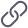https://archive.ics.uci.edu/ml/datasets/heart+disease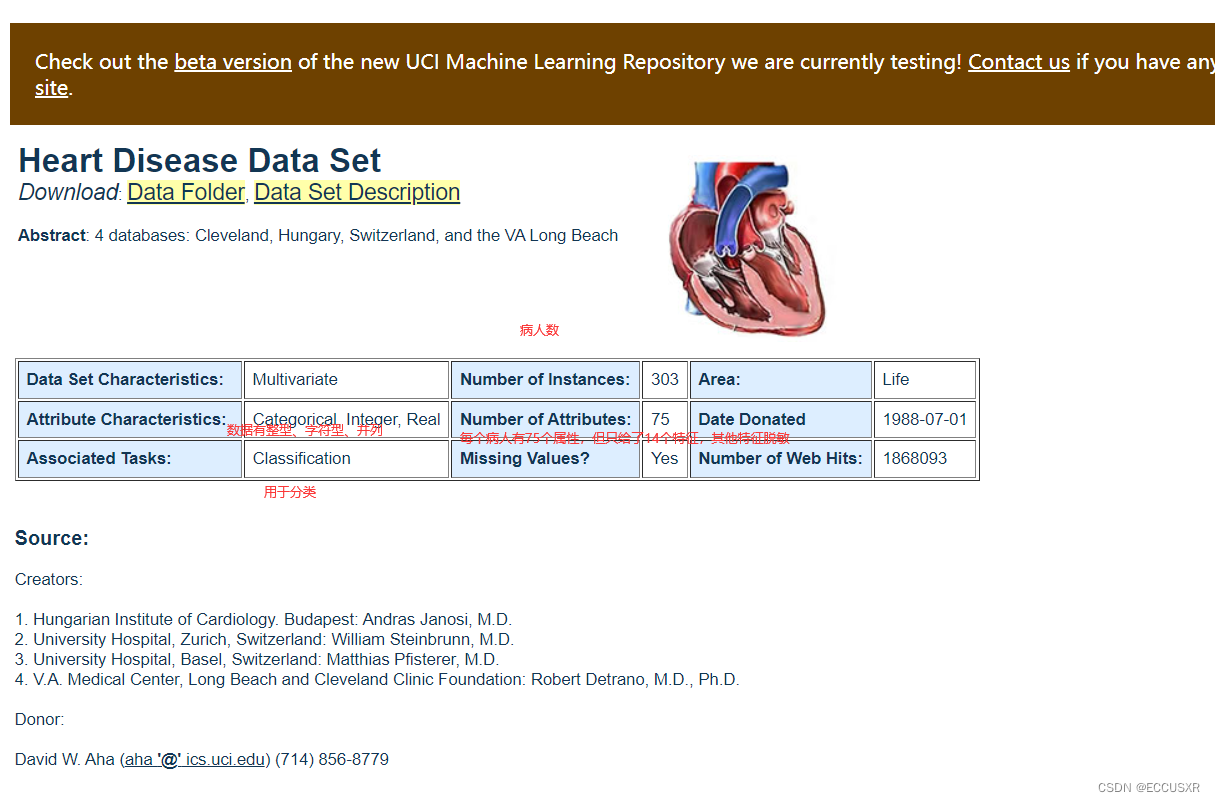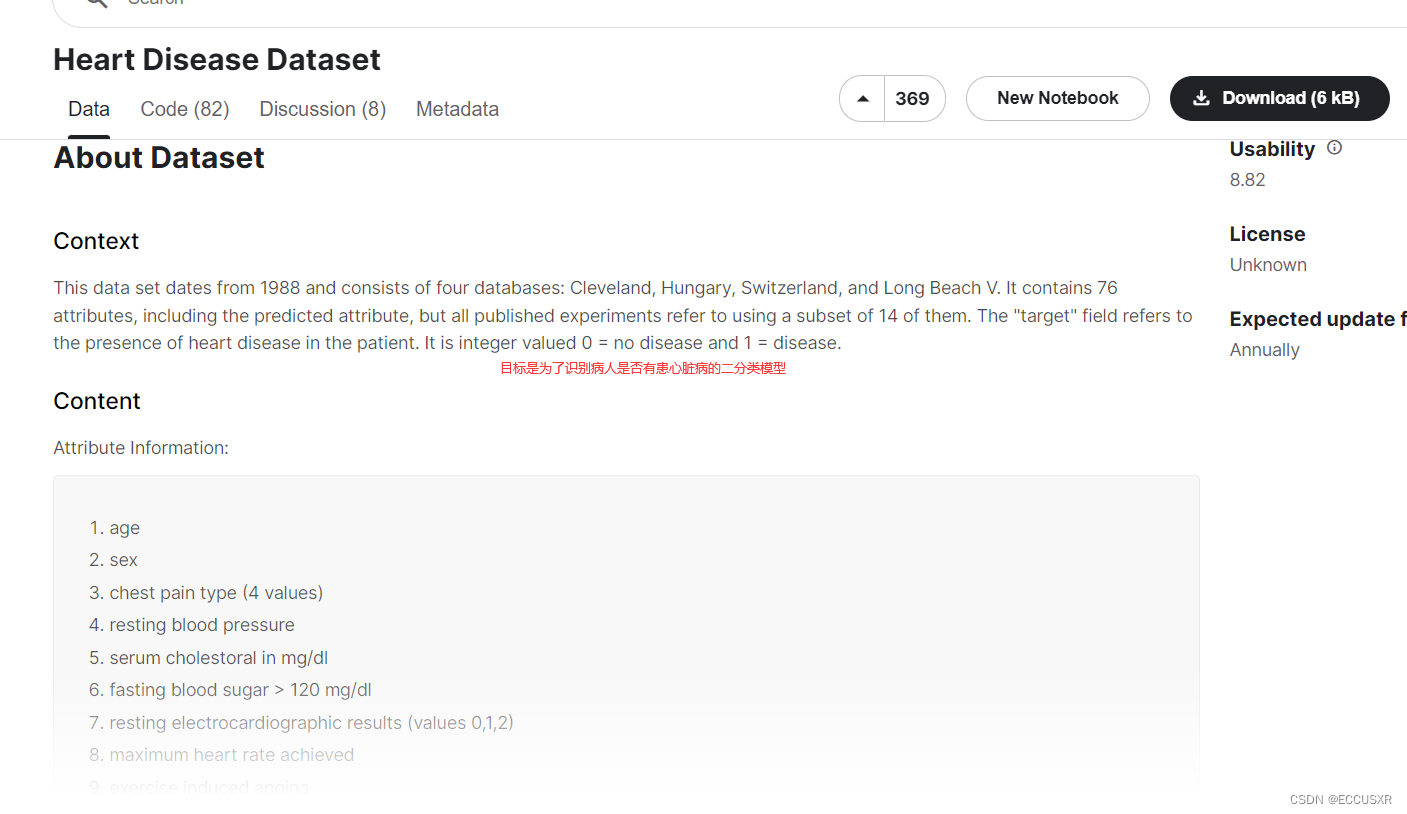得到的csv文件为：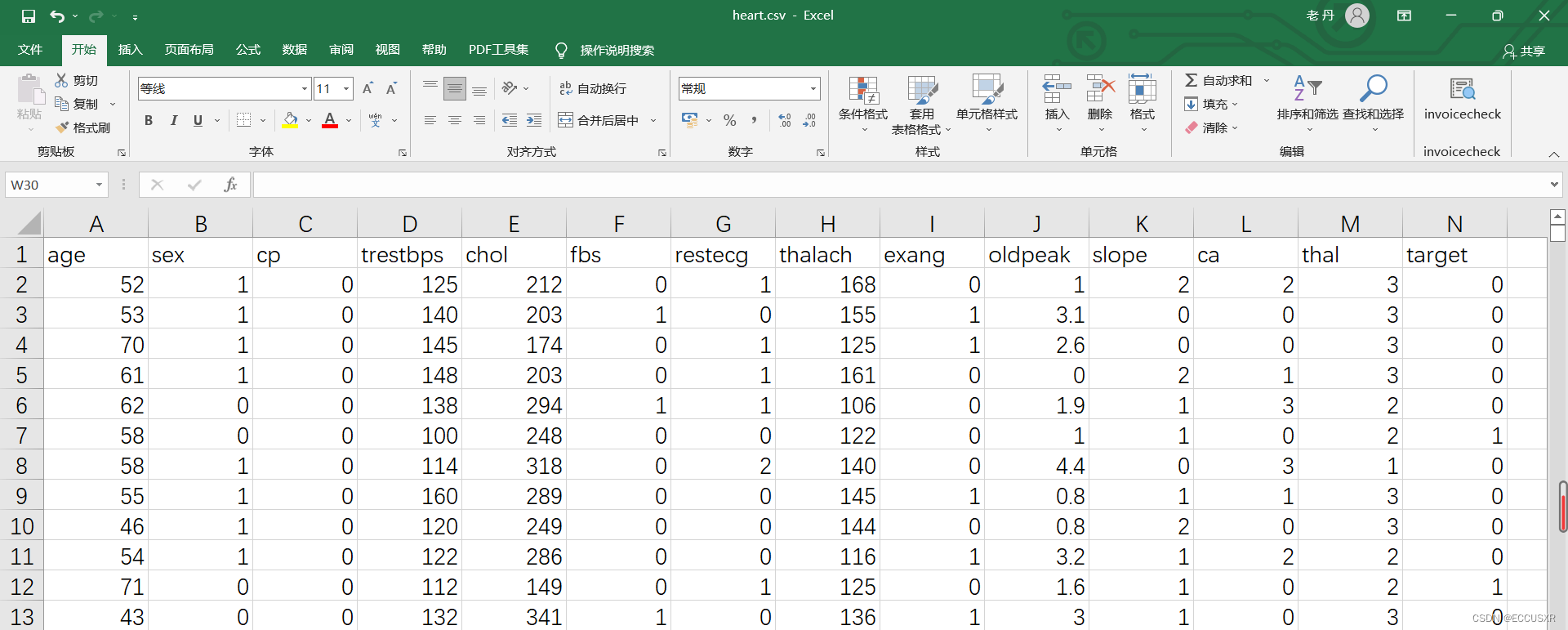## 2. 数据集介绍

数据集有1025行，14列。每行表示一个病人。13列表示特征，1列表示标签(是否患心脏病)

| age      | 年龄                                                         |
| sex      | 性别，1表示男，0表示女                                       |
| cp       | 心绞痛病史，1:典型心绞痛，2:非典型心绞痛，3:无心绞痛，4:无症状 |
| trestbps | 静息血压，入院时测量得到，单位为毫米汞柱(mm Hg)              |
| chol     | 胆固醇含量，单位:mgldl                                       |
| fbs      | 空腹时是否血糖高，如果空腹血糖大于120 mg/dl，值为1，否则值为0 |
| restecg  | 静息时的心电图特征。0:正常。1:  ST-T波有异常。2:根据Estes准则，有潜在的左 |
| thalach  | 最大心率                                                     |
| exang    | 运动是否会导致心绞痛,1表示会，0表示不会                      |
| oldpeak  | 运动相比于静息状态，心电图中的ST-T波是否会被压平。1表示会，0表示不会 |
| slope    | 心电图中ST波峰值的坡度（1:上升，2:平坦，3:下降)              |
| ca       | 心脏周边大血管的个数(0-3)                                    |
| thal     | 是否患有地中海贫血症(3:无，6: fixed defect; 7: reversable defect) |
| target   | 标签列。是否有心脏病，0表示没有，1表示有                     |

## 3. 数据预处理

首先要区分好：定类、定序、定距、定比、四种数据的特征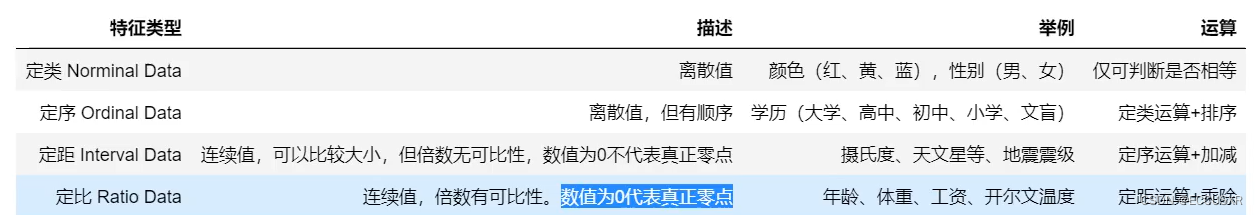① 我们需要将定类特征由整数转为实际对应的字符串，还原为真实含义。

② 将定类数据扩展为特征

③ 导出预处理后的数据

import pandas as pd

# 将定类特征由整数编码转为实际对应的字符串，还原为真实含义
df['sex'][df['sex'] == 0] = 'female'
df['sex'][df['sex'] == 1] = 'male'
df['cp'][df['cp'] == 0] = 'typical angina'
df['cp'][df['cp'] == 1] = 'atypical angina'
df['cp'][df['cp'] == 2] = 'non-anginal pain'
df['cp'][df['cp'] == 3] = 'asymptomatic'

df['fbs'][df['fbs'] == 0] = 'lower than 120mg/ml'
df['fbs'][df['fbs'] == 1] = 'greater than 120mg ml'

df['restecg'][df['restecg'] == 0] = 'normal'
df['restecg'][df['restecg'] == 1] = 'ST-T wave abnormality'
df['restecg'][df['restecg'] == 1] = 'left ventricular hyper trophy'

df['exang'][df['exang'] == 0] = 'no'
df['exang'][df['exang'] == 1] = 'yes'

df['slope'][df['slope'] == 0] = 'upsloping'
df['slope'][df['slope'] == 1] = 'flat'
df['slope'][df['slope'] == 1] = 'downsloping'

df['thal'][df['thal'] == 0] = 'unknown'
df['thal'][df['thal'] == 1] = 'normal'
df['thal'][df['thal'] == 1] = 'fixed defect'
df['thal'][df['thal'] == 1] = 'reversable defect'

# 将离散的定类和定序特征列转为One-Hot独热编码
# 将定类数据扩展为特征
df = pd.get_dummies(df)

# 导出预处理后的数据
df.to_csv('process_heart.csv',index=False)

##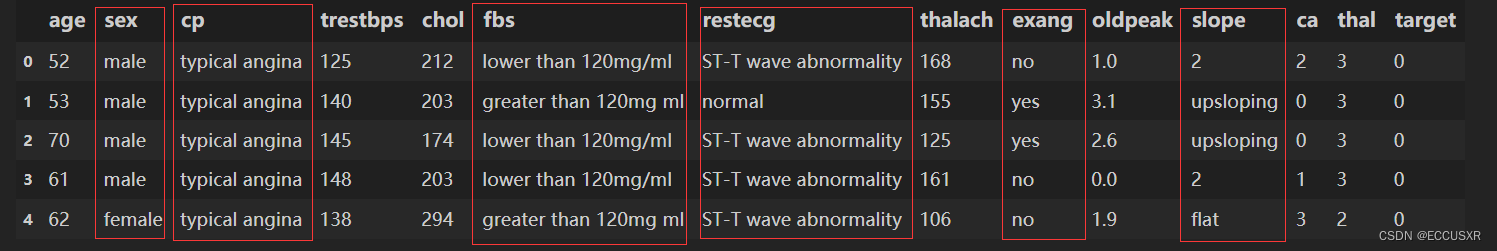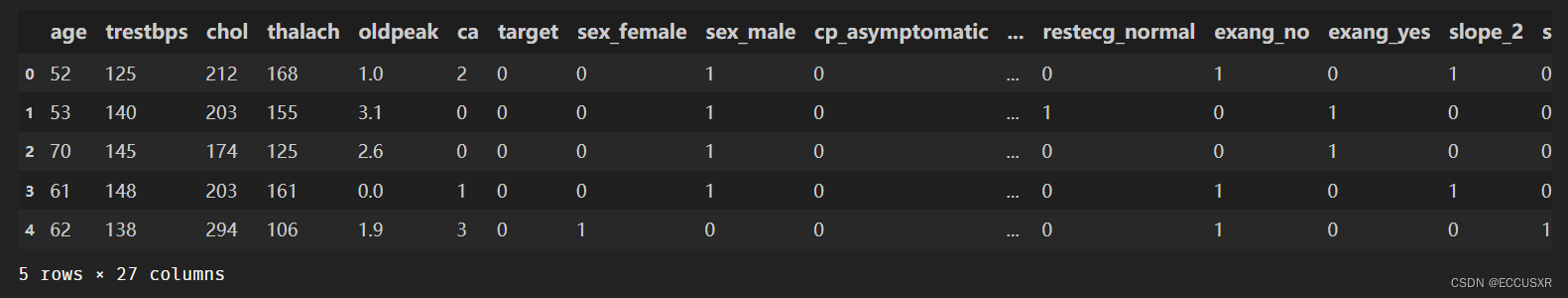## 4. 构建随机森林分类模型

第一，要拿数据与处理后的文件。

import numpy as np
import pandas as pd
import matplotlib.pyplot as p1t
%matplotlib inline
# 忽略警告
import warnings
warnings.filterwarnings("ignore")
df = pd.read_csv('process_heart.csv')

第二，将数据分为输入和输出

# 去掉这一列  矩阵用X表示  input
X = df.drop('target',axis=1)

# y向量
y = df['target']

第三，数据划分为测试集和训练集

# 将数据划分为训练集和测试集,20%作为测试集，随机数种子
from sklearn.model_selection import train_test_split
X_train,X_test,y_train,y_test = train_test_split(X,y,test_size=0.2,random_state=10)

第四，构建随机森林分类模型，在训练集上训练模型

# 构建随机森林分类模型，在训练集上训练模型
from sklearn.ensemble import RandomForestClassifier
# 最大深度为5，决策树为100，随机种子数为5
model = RandomForestClassifier(max_depth=5,n_estimators=100,random_state=5)
# fit 拟合
model.fit(X_train,y_train)
# 可以查看第7个决策树
estimator = model.estimators_

第五，将决策树可视化

# 将输出特征值转为字符串
feature_names = X_train.columns
y_train_str = y_train.astype('str')
y_train_str[y_train=='0'] = 'no disease'
y_train_str[y_train=='1'] = 'disease'
y_train_str = y_train_str.values

# 将决策树可视化
from sklearn.tree import export_graphviz
export_graphviz(estimator,out_file='tree.dot',
feature_names = feature_names,
class_names = y_train_str,
rounded = True,proportion = True,
label='root',
precision = 2,filled = True)

from subprocess import call
call(['dot','-Tpng','tree.dot','-o','tree.png','-Gdpi=600'],shell=True)
from IPython.display import Image
Image(filename = 'tree.png')



## 5. 预测测试集数据

#在训练集上训练得到随机森林模型之后，就可以对测试集上的数据进行预测，也可以对新未知数据进行预测。 将预测结果与测试集真正的标签相比#较，可以定量评估模型指标，绘制混淆矩阵，计算Precision、Recall、F1-Score等评估指标，并绘制ROC曲线。混淆矩阵、ROC曲线、F1-#Score
第一，模型准备


# 忽略警告
import warnings
warnings.filterwarnings("ignore")

import numpy as np
import pandas as pd
import matplotlib.pyplot as plt
%matplotlib inline
#导入数据集,划分特征和标签
X = df.drop('target',axis=1)
y = df['target']
#划分训练集和测试集
from sklearn.model_selection import train_test_split
X_train,X_test,y_train,y_test = train_test_split(X, y, test_size=0.2,random_state=10)

#构建随机森林模型
from sklearn.ensemble import RandomForestClassifier
model = RandomForestClassifier(max_depth=5,n_estimators=100)
model.fit(X_train, y_train)

第二，将其中一个测试样本转成数组的形式


## 对数据进行位置索引，从而在数据表中提取出相应的数据。
X_test.iloc
# 筛选出未知样本
test_sample = X_test.iloc
# 变成二维
test_sample = np.array(test_sample).reshape(1,-1)

第三，预测单个未知样本

#  二分类定性分类结果
model.predict(test_sample)
# 二分类定量分类结果
model.predict_proba(test_sample)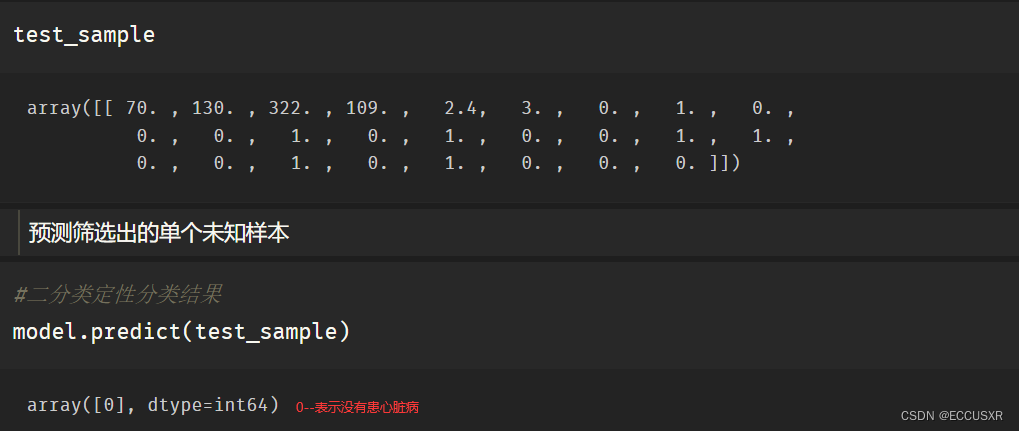第四，预测整个测试样本

y_pred = model.predict(X_test)
# 得到患心脏病和不患心脏病的置信度
y_pred_proba = model.predict_proba(X_test)
# 切片操作 只获得患心脏病的置信度
model.predict_proba(X_test)[:,1]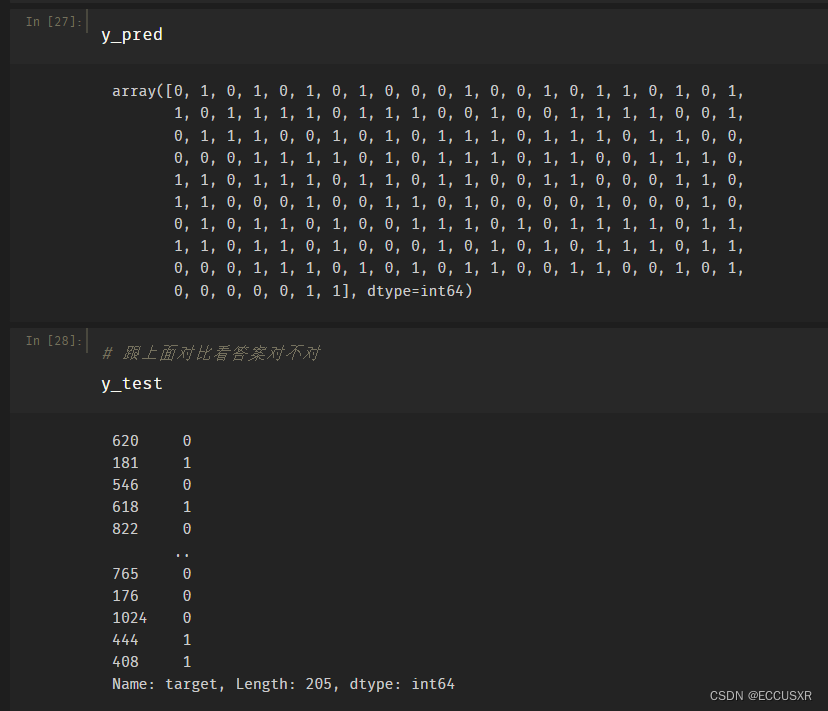## 6. 构建混淆矩阵

# 混淆矩阵
from sklearn.metrics import confusion_matrix
confusion_matrix_model = confusion_matrix(y_test, y_pred)
# 将混淆矩阵绘制出来
import itertools
def cnf_matrix_plotter(cm,classes):
'''
传入混淆矩阵和标签名称列表，绘制混淆矩阵
'''
# plt.imshow (cm, interpolation='nearest', cmap=plt.cm.Greens)
plt.imshow(cm, interpolation='nearest', cmap=plt.cm.Oranges)
plt.title('Confusion Matrix')
plt.colorbar()
tick_marks = np.arange(len(classes))
plt.xticks(tick_marks,classes,rotation=45)
plt.yticks(tick_marks,classes)

threshold = cm.max() / 2.
for i, j in itertools.product(range(cm. shape), range(cm.shape)):
plt.text(j, i, cm[i,j],
horizontalalignment="center",
color="white" if cm[i,j] > threshold else "black",fontsize=25)
plt.tight_layout()
plt.ylabel('True Label')
plt.xlabel(' Predicted Label')
plt.show()
cnf_matrix_plotter(confusion_matrix_model,['Healthy','Disease'])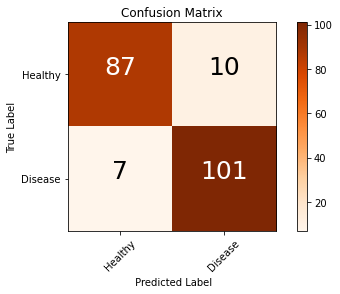## 7. 计算查全率、召回率、调和平均值

# 计算查全率、召回率、调和平均值
from sklearn.metrics import classification_report
print(classification_report(y_test,y_pred,target_names=['Healthy', 'Disease']))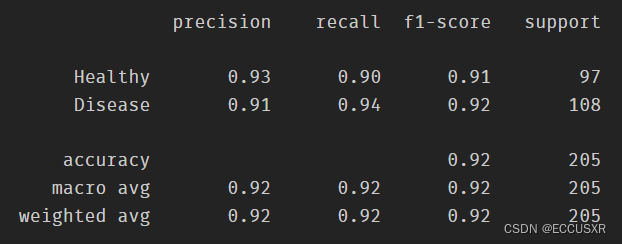## 8. ROC曲线、AUC曲线

# ROC曲线
y_pred_quant = model.predict_proba(X_test)[:,1]
from sklearn. metrics import roc_curve,auc
fpr,tpr,thresholds = roc_curve(y_test,y_pred_quant)
plt.plot(fpr,tpr)
plt.plot([0,1],[0,1],ls="--",c=".3")
plt.xlim([0.0,1.0])
plt.ylim([0.0,1.0])
plt.rcParams['font.size'] = 12
plt.title('ROC curve')
plt.xlabel('False Positive Rate (1 - Specificity)')
plt.ylabel('True Positive Rate (Sensitivity)')
plt.grid(True)

# 计算AUC曲线
auc(fpr,tpr)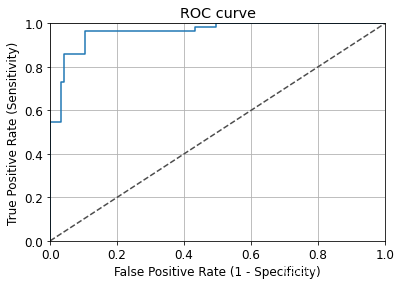展开全文机器学习 人工智能 python sklearn
• 数据集提供了许多变量以及有或不有心脏的目标条件。下面，数据首先用于一个简单的随机森林模型，然后使用 ML 可解释性工具和技术对该模型进行研究。 感兴趣的可以参考原文学习，notebook地址为：...

公众号：尤而小屋
作者：Peter
编辑：Peter

大家好，我是Peter~

新年的第一个项目实践~给大家分享一个新的kaggle案例：基于随机森林模型（RandomForest）的心脏病人预测分类。本文涉及到的知识点主要包含：

• 数据预处理和类型转化

• 随机森林模型建立与解释

• 决策树如何可视化

• 基于混淆矩阵的分类评价指标

• 部分依赖图PDP的绘制和解释

• AutoML机器学习SHAP库的使用和解释（待提升）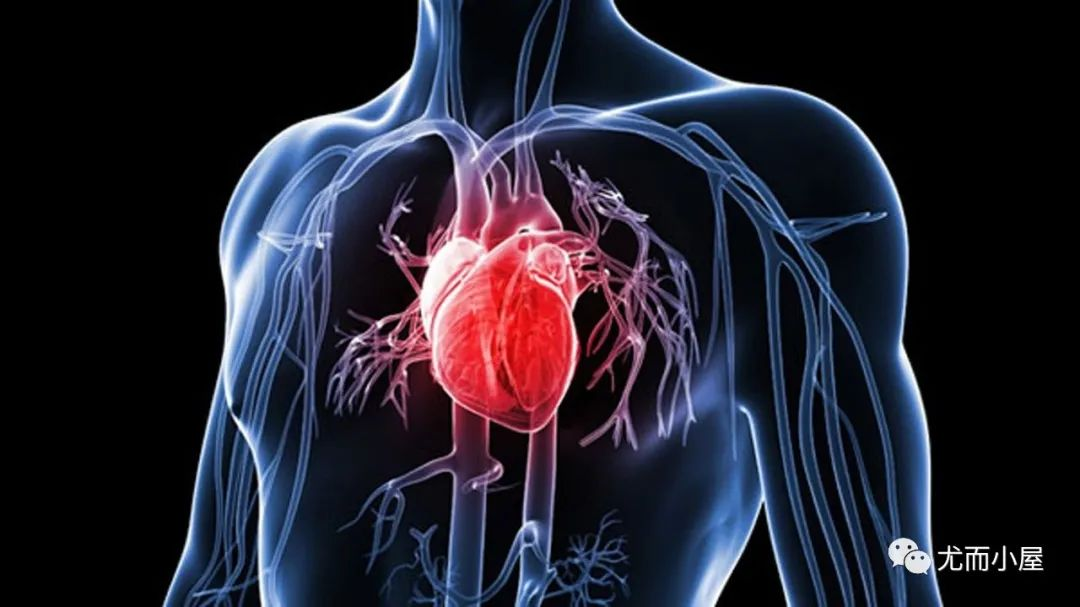## 导读

Of all the applications of machine-learning, diagnosing any serious disease using a black box is always going to be a hard sell. If the output from a model is the particular course of treatment (potentially with side-effects), or surgery, or the absence of treatment, people are going to want to know why.

在机器学习的所有应用中，使用黑匣子诊断任何严重疾病总是很难的。如果模型的输出是特定的治疗过程（可能有副作用）、手术或是否有疗效，人们会想知道为什么。

This dataset gives a number of variables along with a target condition of having or not having heart disease. Below, the data is first used in a simple random forest model, and then the model is investigated using ML explainability tools and techniques.

该数据集提供了许多变量以及患有或不患有心脏病的目标条件。下面，数据首先用于一个简单的随机森林模型，然后使用 ML 可解释性工具和技术对该模型进行研究。

感兴趣的可以参考原文学习，notebook地址为：https://www.kaggle.com/tentotheminus9/what-causes-heart-disease-explaining-the-model

## 导入库

本案例中涉及到多个不同方向的库：

• 数据预处理

• 多种可视化绘图；尤其是shap的可视化，模型可解释性的使用（后面会专门写这个库）

• 随机森林模型

• 模型评价等

import numpy as np
import pandas as pd
import matplotlib.pyplot as plt
import seaborn as sns
from sklearn.ensemble import RandomForestClassifier
from sklearn.tree import DecisionTreeClassifier
from sklearn.tree import export_graphviz
from sklearn.metrics import roc_curve, auc
from sklearn.metrics import classification_report
from sklearn.metrics import confusion_matrix
from sklearn.model_selection import train_test_split
import eli5
from eli5.sklearn import PermutationImportance
import shap
from pdpbox import pdp, info_plots
np.random.seed(123)

pd.options.mode.chained_assignment = None

## 数据探索EDA

1、导入数据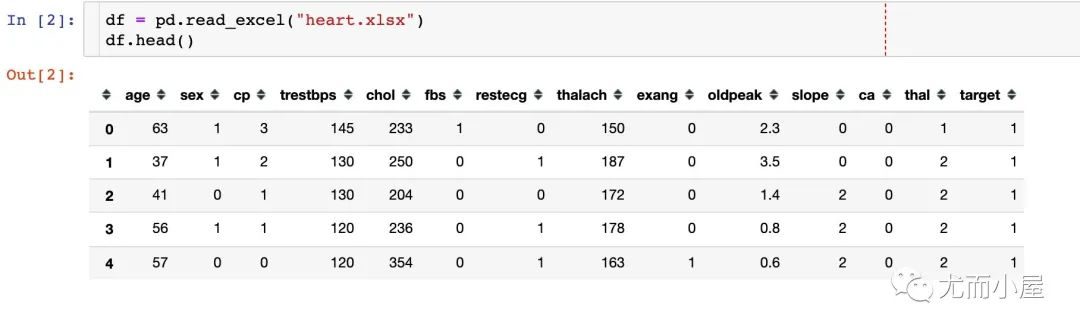2、缺失值情况

数据比较完美，没有任何缺失值！## 字段含义

在这里重点介绍下各个字段的含义。Peter近期导出的数据集中的额字段和原notebook中的字段名字写法稍有差异（时间原因导致），还好Peter已经为大家做了一一对应的关系，下面是具体的中文含义：

1. age：年龄

2. sex 性别 1=male  0=female

3. cp  胸痛类型；4种取值情况

• 1：典型心绞痛

• 2：非典型心绞痛

• 3：非心绞痛

• 4：无症状

trestbps 静息血压

chol 血清胆固醇

fbs 空腹血糖 >120mg/dl  ：1=true；0=false

restecg 静息心电图(值0,1,2)

thalach 达到的最大心率

exang 运动诱发的心绞痛(1=yes;0=no)

oldpeak 相对于休息的运动引起的ST值(ST值与心电图上的位置有关)

slope 运动高峰ST段的坡度

• 1：upsloping向上倾斜

• 2：flat持平

• 3：downsloping向下倾斜

ca  The number of major vessels(血管) (0-3)

thal A blood disorder called thalassemia ，一种叫做地中海贫血的血液疾病(3 = normal；6 = fixed defect;；7 = reversable defect)

target 生病没有(0=no；1=yes)

原notebook中的英文含义；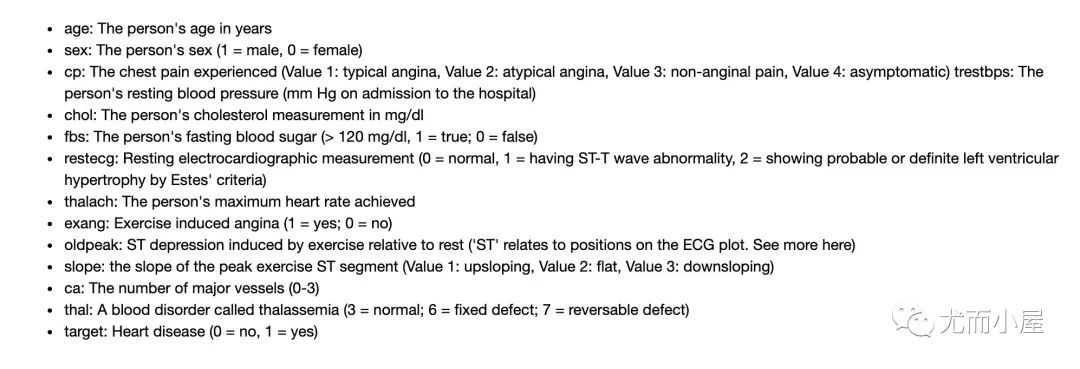下面是Peter整理的对应关系。本文中以当前的版本为标准：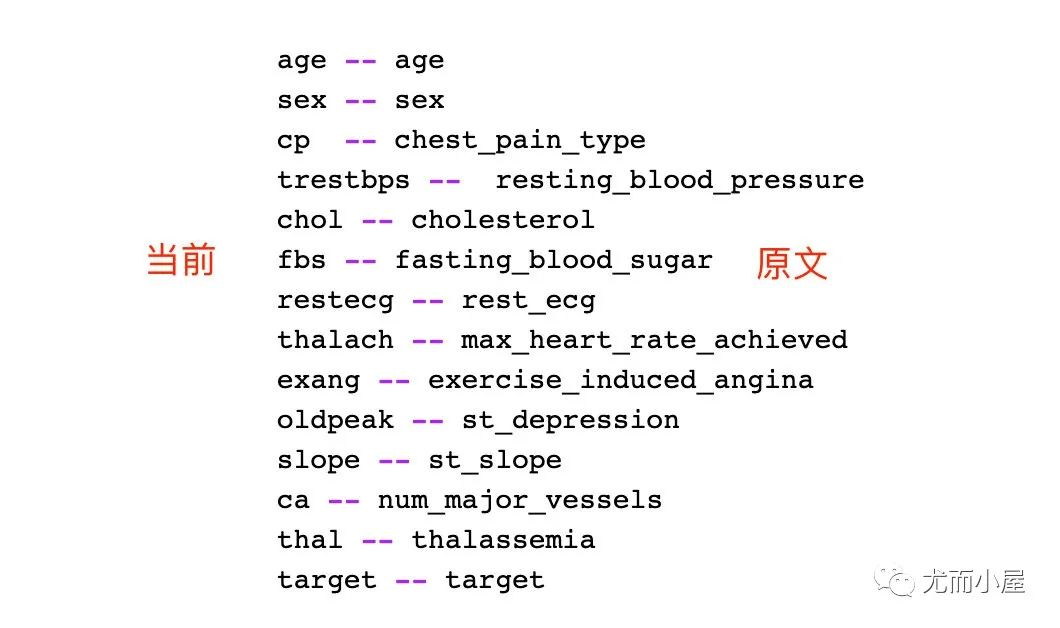## 字段转化

### 转化编码

对部分字段进行一一的转化。以sex字段为例：将数据中的0变成female，1变成male

# 1、sex

df["sex"][df["sex"] == 0] = "female"
df["sex"][df["sex"] == 1] = "male"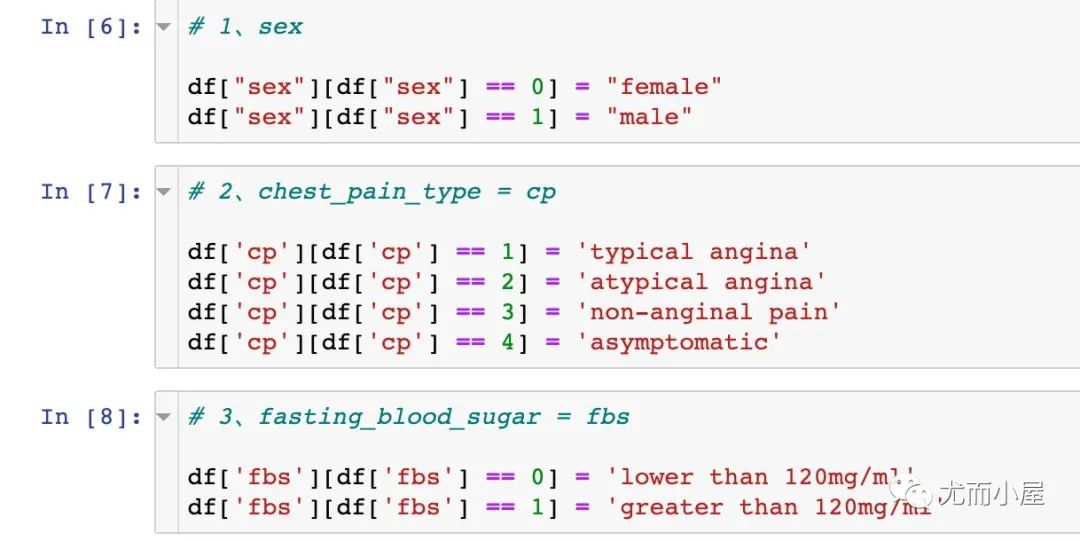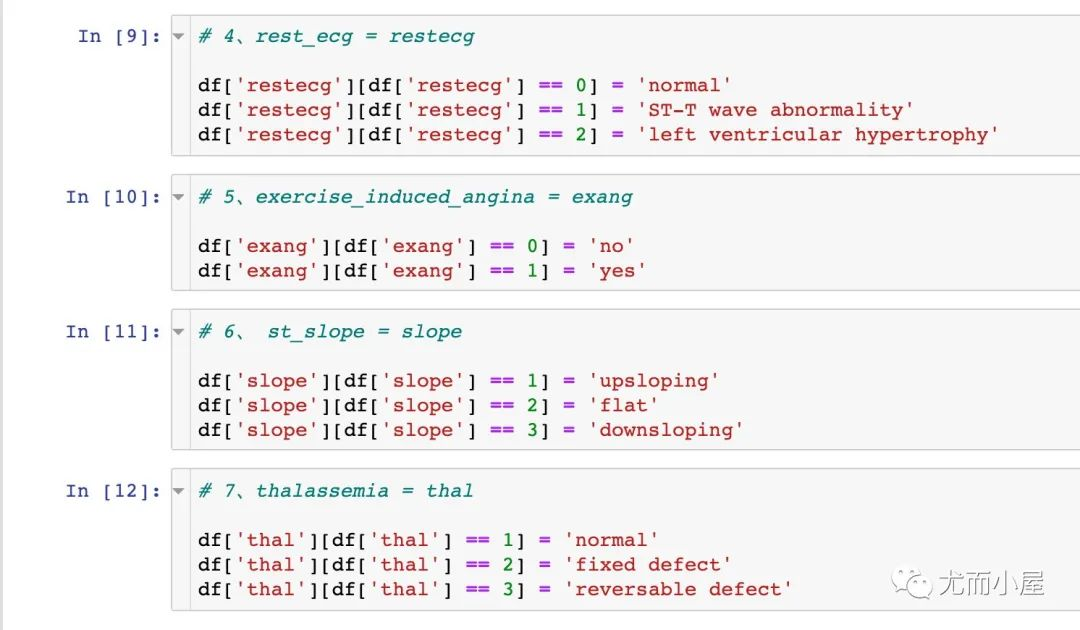### 字段类型转化

# 指定数据类型
df["sex"] = df["sex"].astype("object")
df["cp"] = df["cp"].astype("object")
df["fbs"] = df["fbs"].astype("object")
df["restecg"] = df["restecg"].astype("object")
df["exang"] = df["exang"].astype("object")
df["slope"] = df["slope"].astype("object")
df["thal"] = df["thal"].astype("object")

### 生成哑变量

# 生成哑变量
df = pd.get_dummies(df,drop_first=True)
df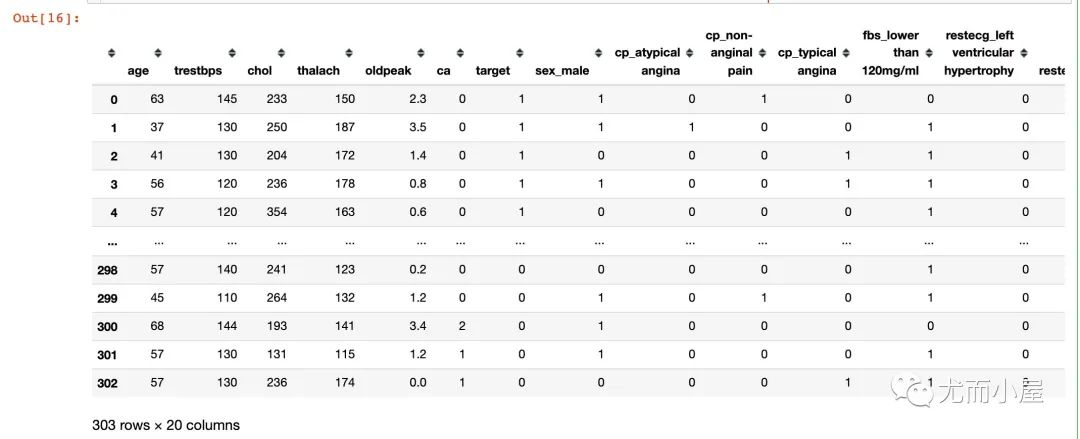## 随机森林RandomForest

### 切分数据

# 生成特征变量数据集和因变量数据集
X = df.drop("target",1)
y = df["target"]

# 切分比例为8：2
X_train, X_test, y_train, y_test = train_test_split(X,y,test_size=0.2,random_state=10)

X_train

### 建模

rf = RandomForestClassifier(max_depth=5)
rf.fit(X_train, y_train)

### 3个重要属性

随机森林中3个重要的属性：

• 查看森林中树的状况：estimators_

• 袋外估计准确率得分：oob_score_，必须是oob_score参数选择True的时候才可用

• 变量的重要性：feature_importances_

### 决策树可视化

在这里我们选择的第二棵树的可视化过程：

# 查看第二棵树的状况
estimator = rf.estimators_

# 全部属性
feature_names = [i for i in X_train.columns]
#print(feature_names)
# 指定数据类型
y_train_str = y_train.astype('str')
# 0-no 1-disease
y_train_str[y_train_str == '0'] = 'no disease'
y_train_str[y_train_str == '1'] = 'disease'
# 训练数据的取值
y_train_str = y_train_str.values
y_train_str[:5]绘图的具体代码为：

# 绘图显示

export_graphviz(
estimator,   # 传入第二颗树
out_file='tree.dot',   # 导出文件名
feature_names = feature_names,  # 属性名
class_names = y_train_str,  # 最终的分类数据
rounded = True,
proportion = True,
label='root',
precision = 2,
filled = True
)

from subprocess import call
call(['dot', '-Tpng', 'tree.dot', '-o', 'tree.png', '-Gdpi=600'])

from IPython.display import Image
Image(filename = 'tree.png')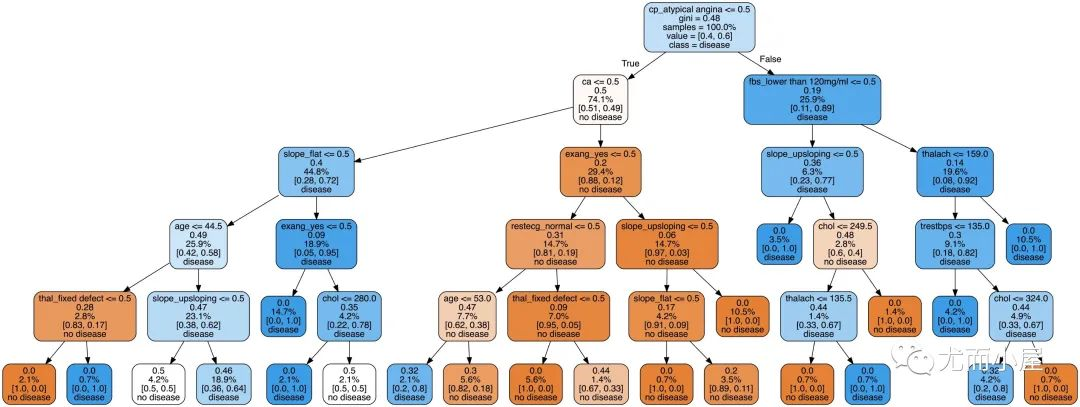决策树的可视化过程能够让我们看到具体的分类过程，但是并不能解决哪些特征或者属性比较重要。后面会对部分属性的特征重要性进行探索

### 模型得分验证

关于混淆矩阵和使用特异性（specificity）以及灵敏度（sensitivity）这两个指标来描述分类器的性能：

# 模型预测
y_predict = rf.predict(X_test)
y_pred_quant = rf.predict_proba(X_test)[:,1]
y_pred_bin = rf.predict(X_test)

# 混淆矩阵
confusion_matrix = confusion_matrix(y_test,y_pred_bin)
confusion_matrix

# 计算sensitivity and specificity
total=sum(sum(confusion_matrix))
sensitivity = confusion_matrix[0,0]/(confusion_matrix[0,0]+confusion_matrix[1,0])
specificity = confusion_matrix[1,1]/(confusion_matrix[1,1]+confusion_matrix[0,1])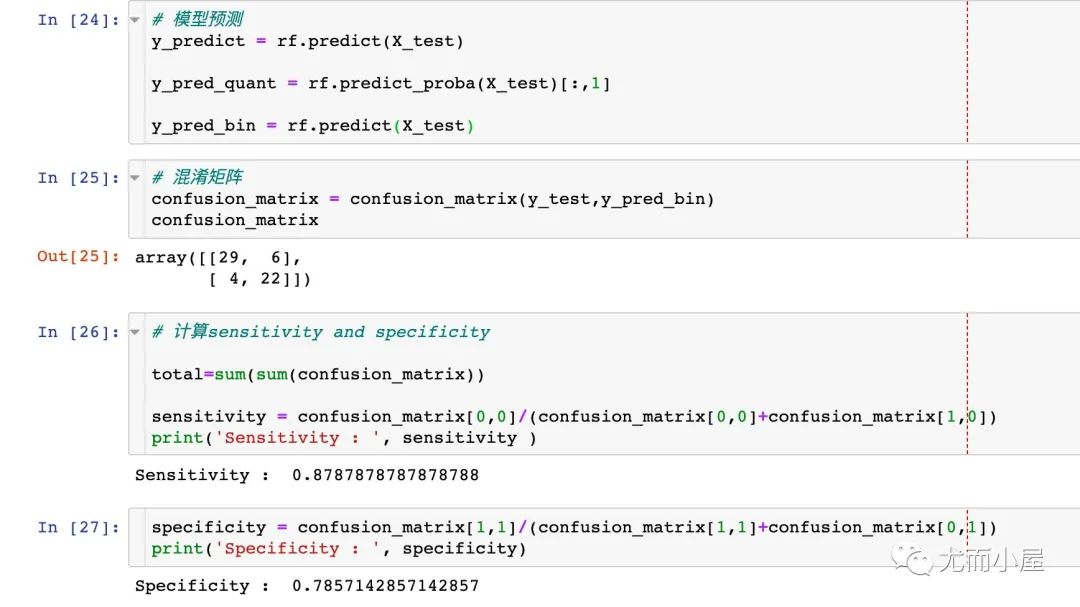### 绘制ROC曲线

fpr, tpr, thresholds = roc_curve(y_test, y_pred_quant)

fig, ax = plt.subplots()
ax.plot(fpr, tpr)

ax.plot([0,1],[0,1],
transform = ax.transAxes,
ls = "--",
c = ".3"
)

plt.xlim([0.0, 1.0])
plt.ylim([0.0, 1.0])

plt.rcParams['font.size'] = 12

# 标题
plt.title('ROC Curve')
# 两个轴的名称
plt.xlabel('False Positive Rate (1 - Specificity)')
plt.ylabel('True Positive Rate (Sensitivity)')
# 网格线
plt.grid(True)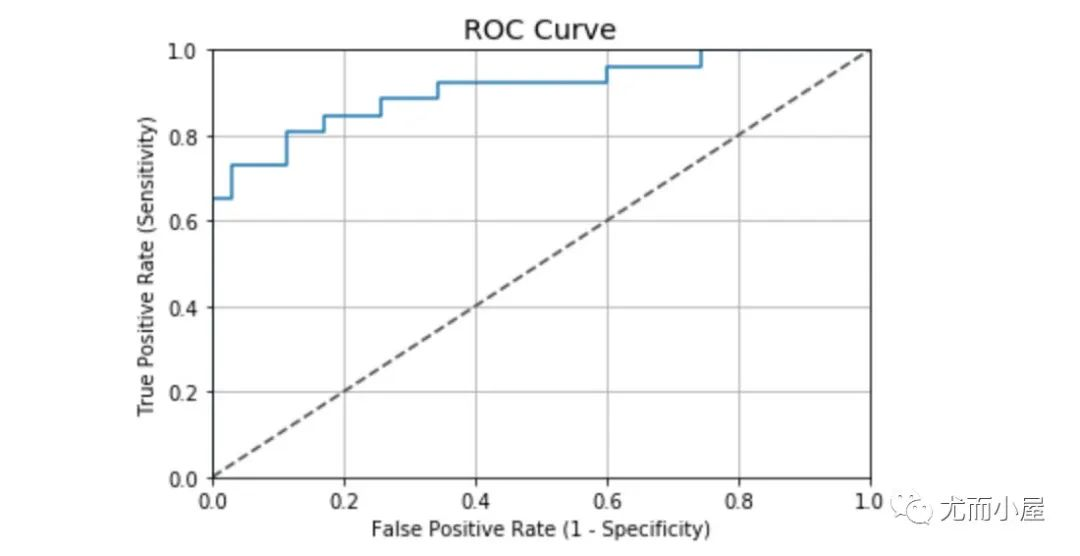本案例中的ROC曲线值：

auc(fpr, tpr)
# 结果
0.9076923076923078

根据一般ROC曲线的评价标准，案例的表现结果还是不错的：

• 0.90 - 1.00 = excellent

• 0.80 - 0.90 = good

• 0.70 - 0.80 = fair

• 0.60 - 0.70 = poor

• 0.50 - 0.60 = fail

### 补充知识点：分类器的评价指标

考虑一个二分类的情况，类别为1和0，我们将1和0分别作为正类（positive）和负类（negative），根据实际的结果和预测的结果，则最终的结果有4种，表格如下：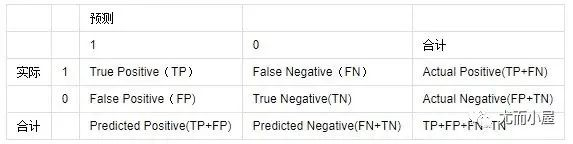常见的评价指标：

1、ACC：classification accuracy，描述分类器的分类准确率

计算公式为：ACC=(TP+TN)/(TP+FP+FN+TN)

2、BER：balanced error rate 计算公式为：BER=1/2*(FPR+FN/(FN+TP))

3、TPR：true positive rate，描述识别出的所有正例占所有正例的比例 计算公式为：TPR=TP/ (TP+ FN)

4、FPR：false positive rate，描述将负例识别为正例的情况占所有负例的比例 计算公式为：FPR= FP / (FP + TN)

5、TNR：true negative rate，描述识别出的负例占所有负例的比例 计算公式为：TNR= TN / (FP + TN)

6、PPV：Positive predictive value计算公式为：PPV=TP / (TP + FP)

7、NPV：Negative predictive value计算公式：NPV=TN / (FN + TN)

其中TPR即为敏感度（sensitivity），TNR即为特异度（specificity）。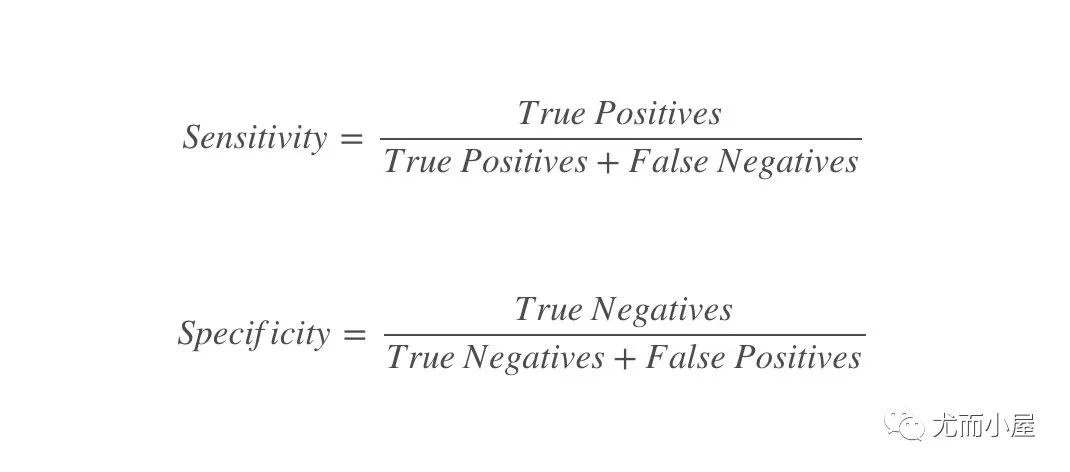来自维基百科的经典图形：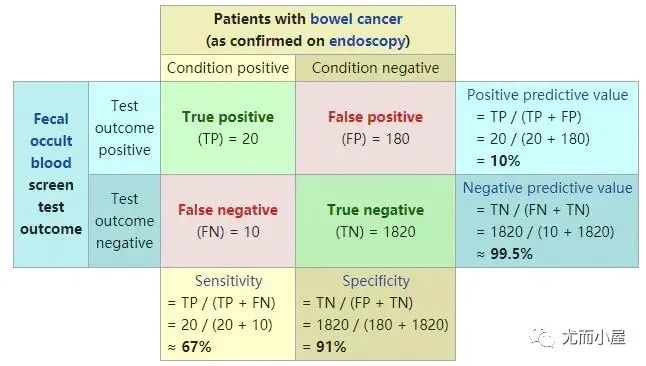## 可解释性

### 排列重要性-Permutation Importance

下面的内容是关于机器学习模型的结果可解释性。首先考察的是每个变量对模型的重要性。重点考量的排列重要性Permutation Importance：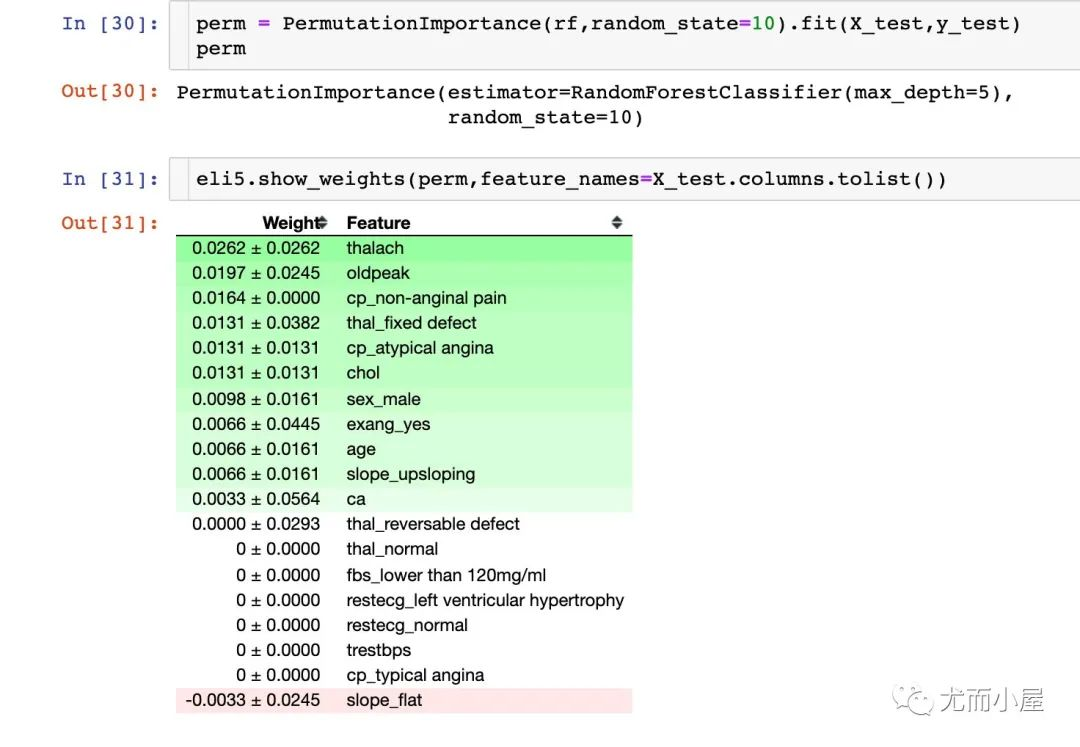## 部分依赖图（ Partial dependence plots ，PDP）

### 一维PDP

Partial Dependence就是用来解释某个特征和目标值y的关系的，一般是通过画出Partial Dependence Plot(PDP)来体现。也就是说PDP在X1的值，就是把训练集中第一个变量换成X1之后，原模型预测出来的平均值。

重点：查看单个特征和目标值的关系

### 字段ca

base_features = df.columns.values.tolist()
base_features.remove("target")

feat_name = 'ca'  # ca-num_major_vessels 原文
pdp_dist = pdp.pdp_isolate(
model=rf,  # 模型
dataset=X_test,  # 测试集
model_features=base_features,  # 特征变量；除去目标值
feature=feat_name  # 指定单个字段
)

pdp.pdp_plot(pdp_dist, feat_name)  # 传入两个参数
plt.show()

通过下面的图形我们观察到：当ca字段增加的时候，患病的几率在下降。ca字段的含义是血管数量（num_major_vessels），也就是说当血管数量增加的时候，患病率随之降低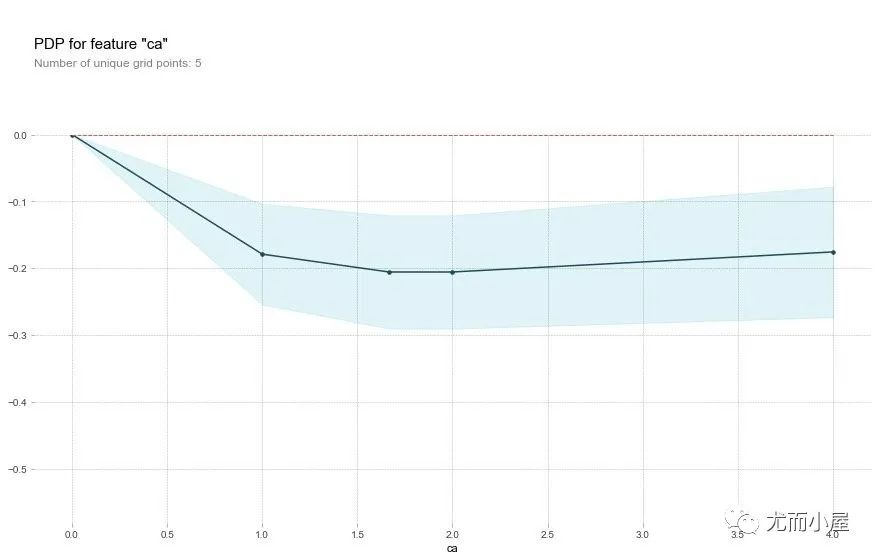### 字段age

feat_name = 'age'

pdp_dist = pdp.pdp_isolate(
model=rf,
dataset=X_test,
model_features=base_features,
feature=feat_name)

pdp.pdp_plot(pdp_dist, feat_name)
plt.show()

关于年龄字段age，原文的描述：

That's a bit odd. The higher the age, the lower the chance of heart disease? Althought the blue confidence regions show that this might not be true (the red baseline is within the blue zone).

翻译：这有点奇怪。年龄越大，患心脏病的几率越低？尽管蓝色置信区间表明这可能不是真的（红色基线在蓝色区域内）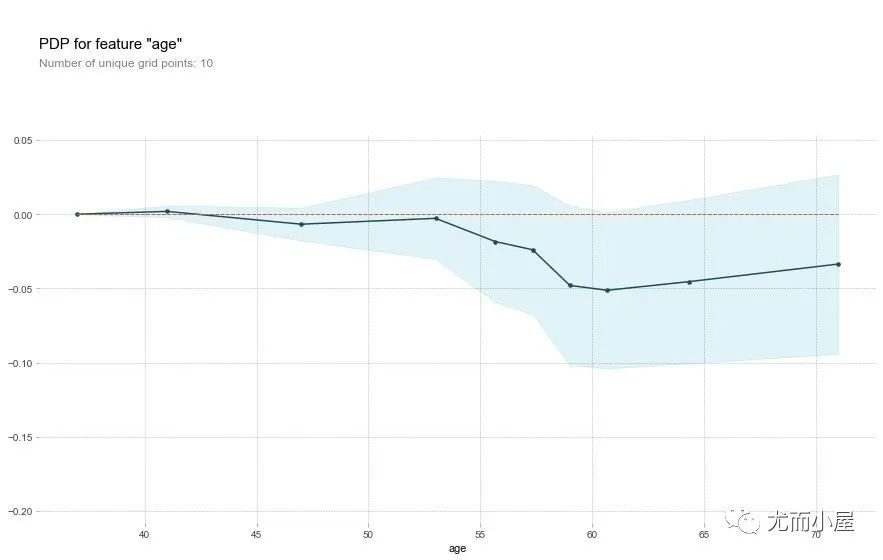### 字段oldpeak

feat_name = 'oldpeak'

pdp_dist = pdp.pdp_isolate(
model=rf,
dataset=X_test,
model_features=base_features,
feature=feat_name)

pdp.pdp_plot(pdp_dist, feat_name)
plt.show()

oldpeak字段同样表明：取值越大，患病几率越低。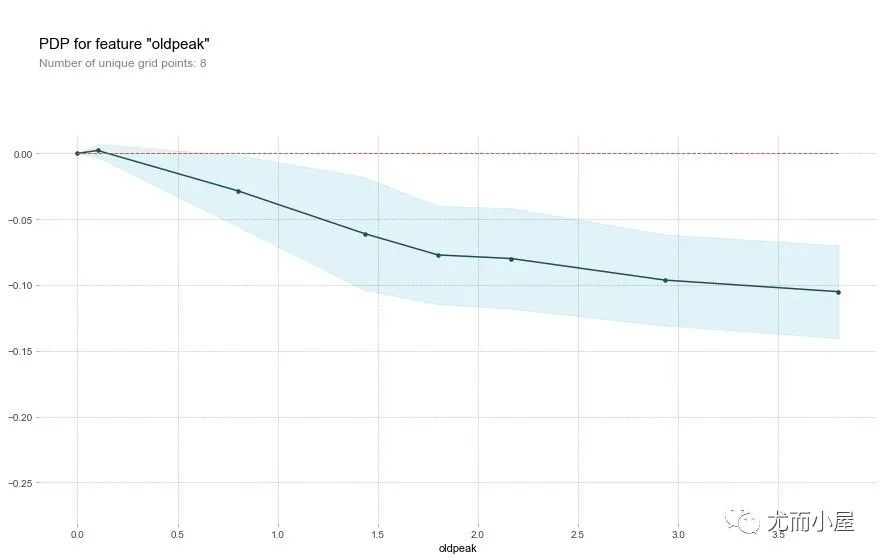这个变量称之为“相对休息运动引起的ST压低值”。正常的状态下，该值越高，患病几率越高。但是上面的图像却显示了相反的结果。

作者推断：造成这个结果的原因除了the depression amount，可能还和slope type有关系。原文摘录如下，于是作者绘制了2D-PDP图形

Perhaps it's not just the depression amount that's important, but the interaction with the slope type? Let's check with a 2D PDP

### 2D-PDP图

查看的是 slope_upsloping 、slope_flat和 oldpeak的关系：

inter1  =  pdp.pdp_interact(
model=rf,  # 模型
dataset=X_test,  # 特征数据集
model_features=base_features,  # 特征
features=['slope_upsloping', 'oldpeak'])

pdp.pdp_interact_plot(
pdp_interact_out=inter1,
feature_names=['slope_upsloping', 'oldpeak'],
plot_type='contour')
plt.show()

## ------------

inter1  =  pdp.pdp_interact(
model=rf,
dataset=X_test,
model_features=base_features,
features=['slope_flat', 'oldpeak']
)

pdp.pdp_interact_plot(
pdp_interact_out=inter1,
feature_names=['slope_flat', 'oldpeak'],
plot_type='contour')
plt.show()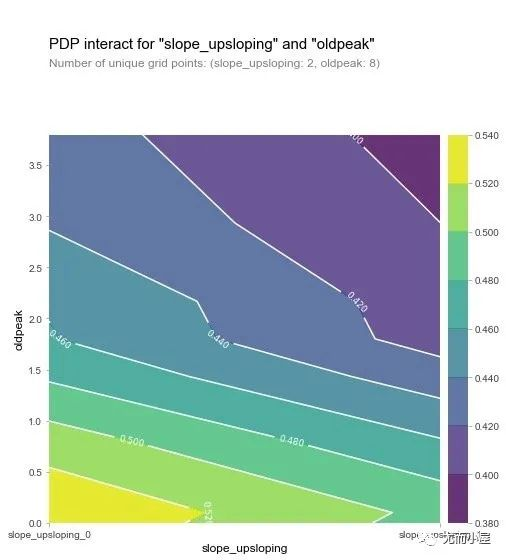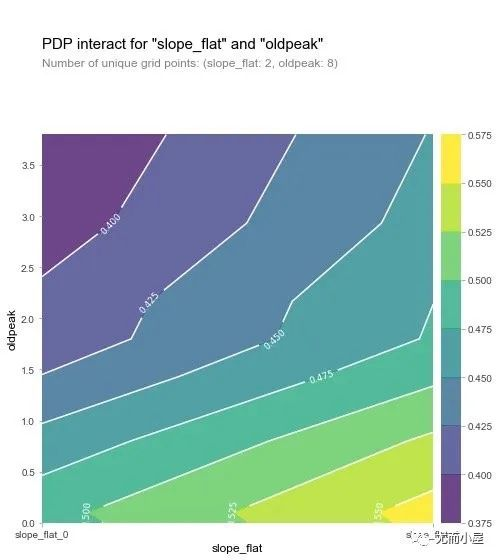从两张图形中我们可以观察到：在oldpeak取值较低的时候，患病几率都比较高（黄色），这是一个奇怪的现象。于是作者进行了如下的SHAP可视化探索：针对单个变量进行分析。

## SHAP可视化

关于SHAP的介绍可以参考文章：https://zhuanlan.zhihu.com/p/83412330    和   https://blog.csdn.net/sinat_26917383/article/details/115400327

SHAP是Python开发的一个"模型解释"包，可以解释任何机器学习模型的输出。下面SHAP使用的部分功能：

### Explainer

在SHAP中进行模型解释之前需要先创建一个explainer，SHAP支持很多类型的explainer，例如deep, gradient, kernel, linear, tree, sampling)。在这个案例我们以tree为例：

# 传入随机森林模型rf
explainer = shap.TreeExplainer(rf)
# 在explainer中传入特征值的数据，计算shap值
shap_values = explainer.shap_values(X_test)
shap_values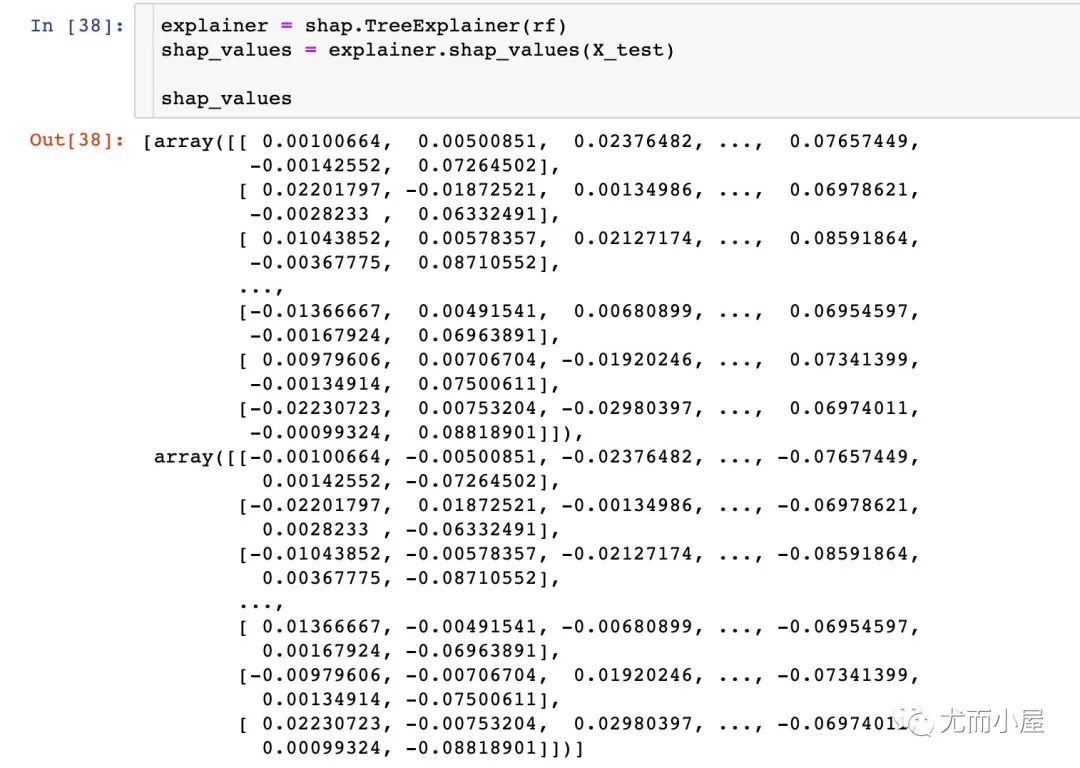### Feature Importance

取每个特征SHAP值的绝对值的平均值作为该特征的重要性，得到一个标准的条形图(multi-class则生成堆叠的条形图：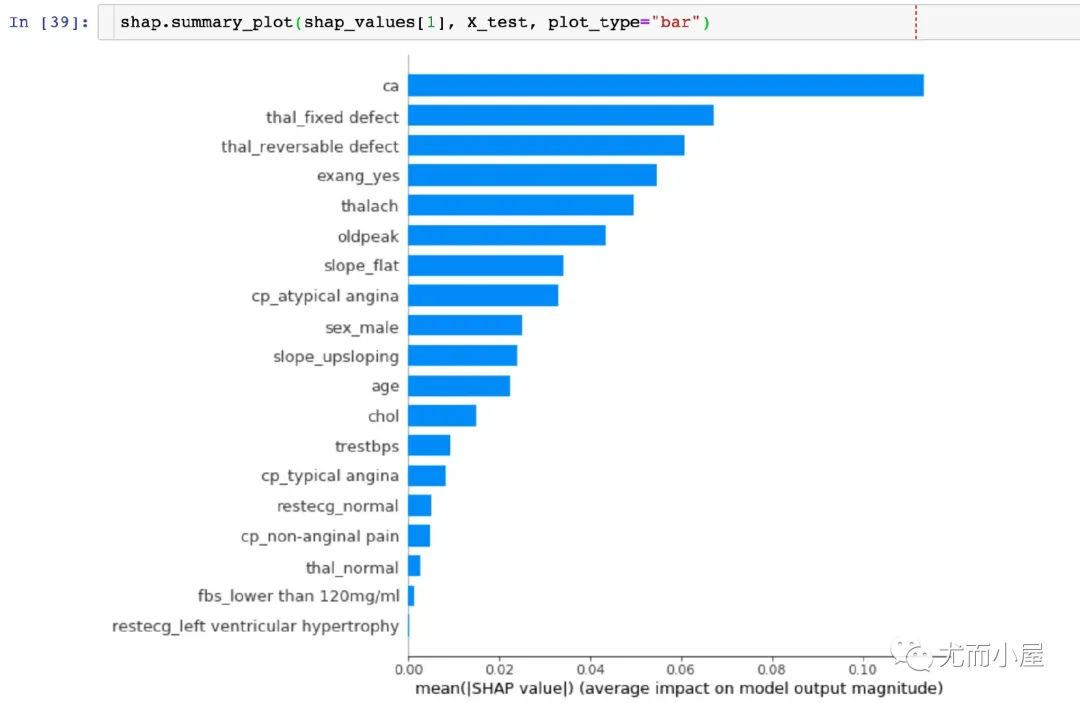结论：能够直观地观察到ca字段的SHAP值最高

### summary_plot

summary plot 为每个样本绘制其每个特征的SHAP值，这可以更好地理解整体模式，并允许发现预测异常值。

• 每一行代表一个特征，横坐标为SHAP值

• 一个点代表一个样本，颜色表示特征值的高低(红色高，蓝色低)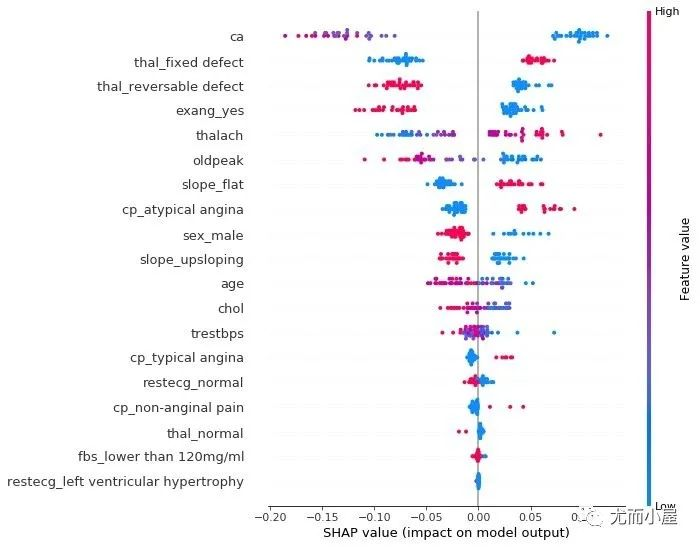### 个体差异

查看单个病人的不同特征属性对其结果的影响，原文描述为：

Next, let's pick out individual patients and see how the different variables are affecting their outcomes

def heart_disease_risk_factors(model, patient):

explainer = shap.TreeExplainer(model)  # 建立explainer
shap_values = explainer.shap_values(patient)  # 计算shape值
shap.initjs()
return shap.force_plot(
explainer.expected_value,
shap_values,
patient)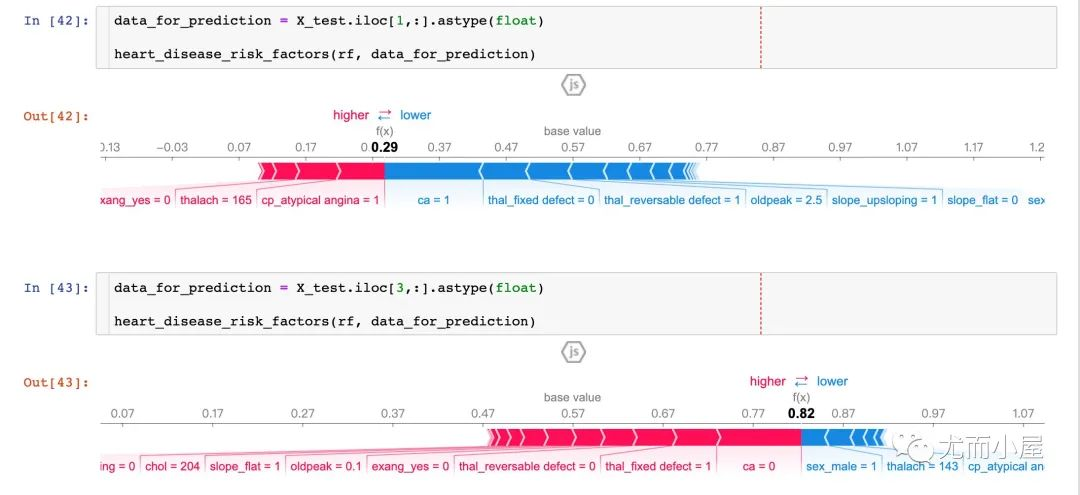从两个病人的结果中显示：

• P1：预测准确率高达29%（baseline是57%），更多的因素集中在ca、thal_fixed_defect、oldpeak等蓝色部分。

• P3：预测准确率高达82%，更多的影响因素在sel_male=0，thalach=143等

通过对比不同的患者，我们是可以观察到不同病人之间的预测率和主要影响因素。

### dependence_plot

为了理解单个feature如何影响模型的输出，我们可以将该feature的SHAP值与数据集中所有样本的feature值进行比较：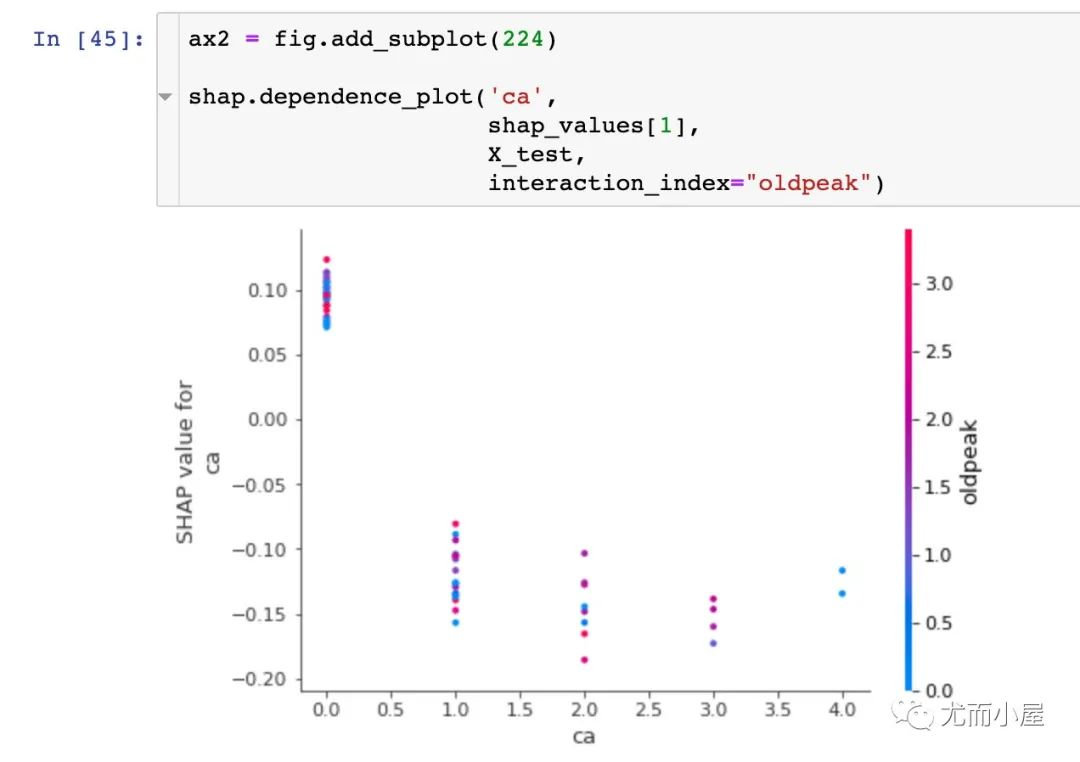### 多样本可视化探索

下面的图形是针对多患者的预测和影响因素的探索。在jupyter notebook的交互式作用下，能够观察不同的特征属性对前50个患者的影响：

shap_values = explainer.shap_values(X_train.iloc[:50])
shap.force_plot(explainer.expected_value,
shap_values,
X_test.iloc[:50])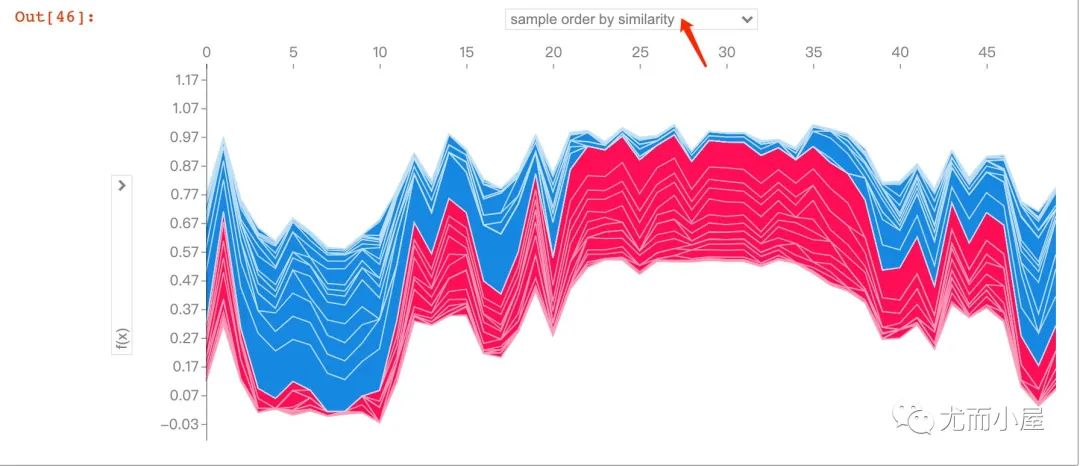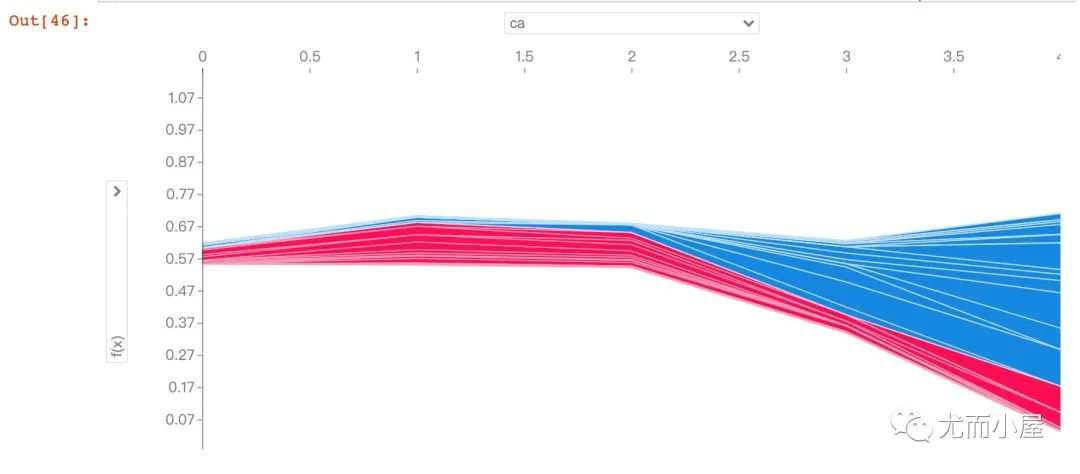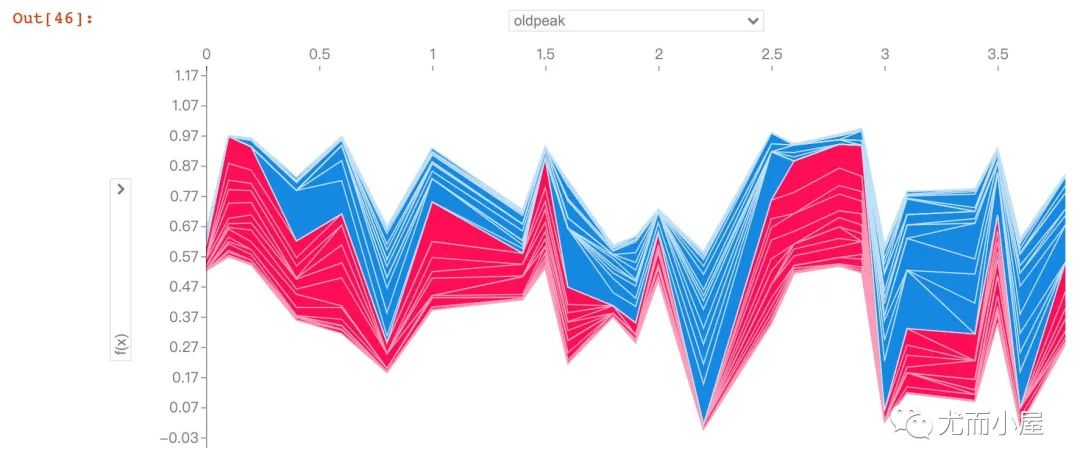数据集获取方式：公众号后台，回复  HeartDisease  即可领取~

干货学习，点赞三连↓
展开全文python 机器学习 人工智能
• 点击上方“Python爬虫与数据挖掘”，进行关注回复“书籍”即可获赠Python从入门到进阶共10本电子书今日鸡汤原得此生长报国，何须生入玉门关。我们都很害怕生病，但感冒发烧这种从小到...

点击上方“Python爬虫与数据挖掘”，进行关注

回复“书籍”即可获赠Python从入门到进阶共10本电子书

原得此生长报国，何须生入玉门关。

我们都很害怕生病，但感冒发烧这种从小到大的疾病我们已经麻木了，因为一星期他就会好，但是随着长大，各种发炎、三高、心脏病、冠心病响应而生。

心脏病作为一种发作起来让人看了就觉得恐怖的疾病，每年不知道夺走多少生命。而那些患病健在的人们也必须在自己后续的生命里割舍太多东西，以防止心脏病发作。

没有得病的时候，我们永远觉得它离自己很远。我对心脏病的认知就是这样，我不知道它患病的原因，也不知哪些原因会引起心脏病。而患病后如何保持正常生活等等，一概不知。

今天在kaggle上看到一个心脏病数据（数据集下载地址和源码见文末），那么借此深入分析一下。

## 数据集读取与简单描述

首先导入library和设置好超参数，方便后续分析。

import numpy as np
import pandas as pd
import matplotlib.pyplot as plt
import seaborn as sns


通过对数据集读取和描述可以得到这两个表格：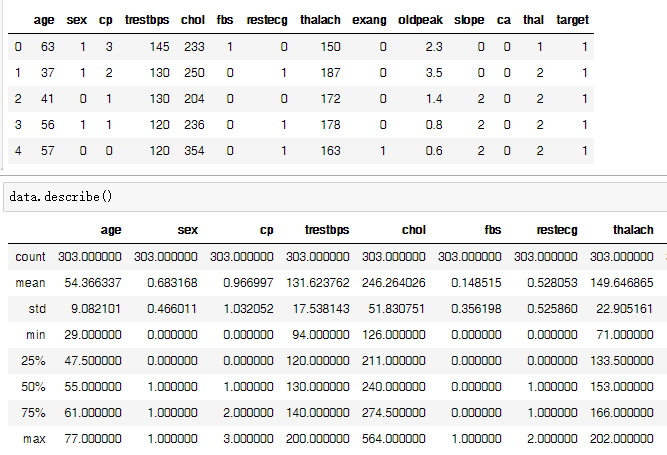可以看到有303行14列数据，每列的标题是age、sex、cp、……、target。他们就像每次去医院的化验单，非专业人士很多都不认识。所以利用官方的解释翻译后含义如下：

• age: 该朋友的年龄

• sex: 该朋友的性别 (1 = 男性, 0 = 女性)

• cp: 经历过的胸痛类型（值1：典型心绞痛，值2：非典型性心绞痛，值3：非心绞痛，值4：无症状）

• trestbps: 该朋友的静息血压（入院时的毫米汞柱）

• chol: 该朋友的胆固醇测量值，单位 ：mg/dl

• fbs: 人的空腹血糖（> 120 mg/dl，1=真；0=假）

• restecg: 静息心电图测量（0=正常，1=患有ST-T波异常，2=根据Estes的标准显示可能或确定的左心室肥大）

• thalach: 这朋友达到的最大心率

• exang: 运动引起的心绞痛（1=有过；0=没有）

• oldpeak: ST抑制，由运动引起的相对于休息引起的（“ ST”与ECG图上的位置有关。这块比较专业，可以点这个看一个解读）

• slope: 最高运动ST段的斜率（值1:上坡，值2:平坦，值3:下坡）

• ca: 萤光显色的主要血管数目（0-4）

• thal: 一种称为地中海贫血的血液疾病（3=正常；6=固定缺陷；7=可逆缺陷）

• target: 心脏病（0=否，1=是）

所以这些信息里都是患病或者健康者的一些身体指标，并没有和他是否抽烟、是否熬夜、是否遗传、是否作息规律那些东西，因此找不到指导现在我们生活的点，比如说明要戒烟戒酒那些东西。

顺手送上一篇知乎链接 此外上边只是我通过原版数据集给的解读翻译的，如有出错误，欢迎纠正

拿到一套数据首先是要看看这个数据大概面貌~

## 男女比例

先看看患病比率，男女比例这些常规的

countNoDisease = len(data[data.target == 0])
countHaveDisease = len(data[data.target == 1])
countfemale = len(data[data.sex == 0])
countmale = len(data[data.sex == 1])
print(f'没患病人数:{countNoDisease }',end=' ,')
print("没有得心脏病比率: {:.2f}%".format((countNoDisease / (len(data.target))*100)))
print(f'有患病人数:{countHaveDisease }',end=' ,')
print("患有心脏病比率: {:.2f}%".format((countHaveDisease / (len(data.target))*100)))
print(f'女性人数:{countfemale }',end=' ,')
print("女性比例: {:.2f}%".format((countfemale / (len(data.sex))*100)))
print(f'男性人数:{countmale }',end=' ,')
print("男性比例: {:.2f}%".format((countmale   / (len(data.sex))*100)))


上边代码得到的答案如下，乍看上去男的多于女的，但前提是这个数据只是这个300人的样本展示，不代表全人类

没患病人数:138 ,没有得心脏病比率: 45.54%
有患病人数:165 ,患有心脏病比率: 54.46%
女性人数:96 ,女性比例: 31.68%
男性人数:207 ,男性比例: 68.32%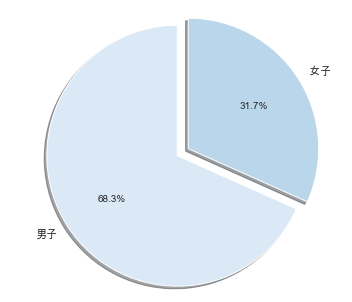除了用饼图看这个面貌，还可以同时看一下

fig, ax =plt.subplots(1,3)  #2个子区域
fig.set_size_inches(w=15,h=5)   # 设置画布大小
sns.countplot(x="sex", data=data,ax=ax)
plt.xlabel("性别 (0 = female, 1= male)")
sns.countplot(x="target", data=data,ax=ax)
plt.xlabel("是否患病 (0 = 未患病, 1= 患病)")
sns.swarmplot(x='sex',y='age',hue='target',data=data,ax=ax)
plt.xlabel("性别 (0 = female, 1= male)")
plt.show()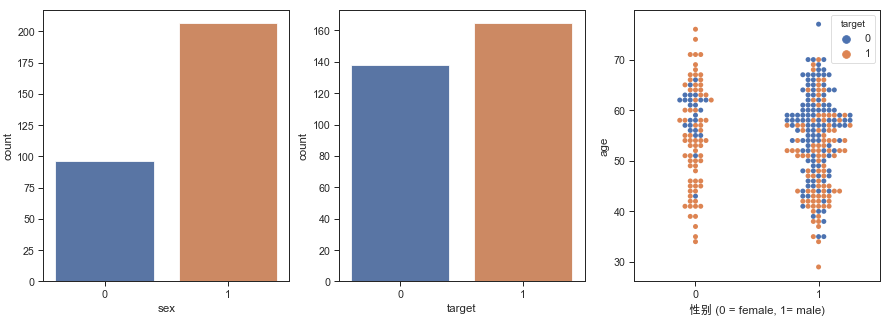从这三联图可以看到男性1多余女性0，患病target1多于未患病0，在年龄分布提琴图里可以看到女性患者比例多于男性患者比例。

其中比列详细拆解一下，见下方代码和图示：

pd.crosstab(data.sex,data.target).plot(kind="bar",figsize=(15,6),color=['#30A9DE','#EFDC05' ])
plt.title('各性别下患病图示')
plt.xlabel('性别 (0 = 女性, 1 = 男性)')
plt.xticks(rotation=0)
plt.legend(["未患病", "患有心脏病"])
plt.ylabel('人数')
plt.show()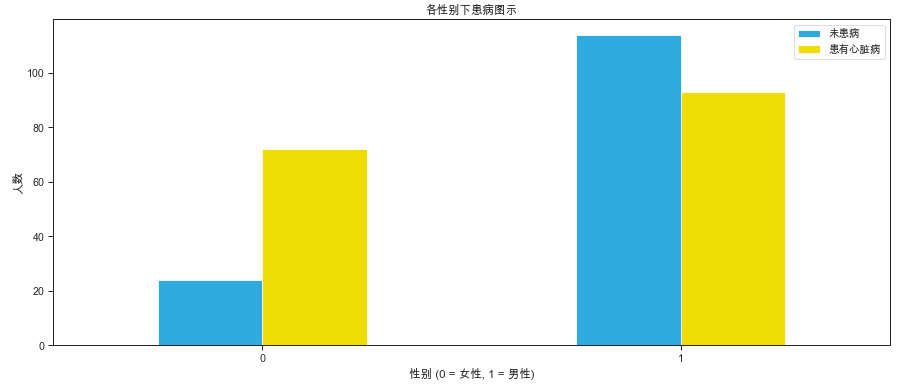在这个数据集中，男性多于女性一倍，分别207和96人；患病患者稍微多余未患病患者，患病165，138人。因为年龄可能是连续的，因此在第三幅图做年龄、性别、患病关系图，单从颜色观察可发现在这个数据集中，女性患病率大于男性。通过第四图和统计可以计算得到，男性患病率44.9% ，女性患病率75%。

需要注意，本文得到的患病率只是这个数据集的。

## 年龄和患病关系

通过以下代码来看一看：随着年龄增长患病比率有没有变化

（现在写这个文章的时候我才想到，可能即使有变化也没有意义，还是样本有限，如果这个样本空间覆盖再提升1000倍才能说明一些问题吧——即年龄和患有心脏病的关系）

pd.crosstab(data.age,data.target).plot(kind="bar",figsize=(25,8))
plt.title('患病变化随年龄分布图')
plt.xlabel('岁数')
plt.ylabel('比率')
plt.savefig('heartDiseaseAndAges.png')
plt.show()


输出的图像如下：就这张图来说37-54岁患病人数多于未患病人数，年龄再继续升高后有没有这个规律了，在70+岁后患病人数又增加，这条仅能作为数据展示，不能作为结论。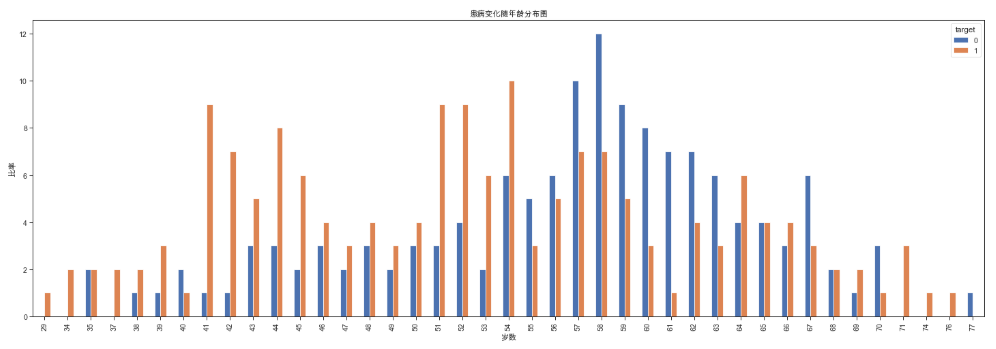数据集中还有很多维度可以组合分析，下边开始进行组合式探索分析

## 年龄-心率-患病三者关系

在这个数据集中，心率的词是‘thalach’，所以看年龄、心率、是否患病的关系。

# 散点图
plt.scatter(x=data.age[data.target==1], y=data.thalach[(data.target==1)], c="red")
plt.scatter(x=data.age[data.target==0], y=data.thalach[(data.target==0)], c='#41D3BD')
plt.legend(["患病", "未患病"])
plt.xlabel("年龄")
plt.ylabel("最大心率")
plt.show()
# 再画个提琴图
sns.violinplot(x=data.target,y=data.trestbps,data=data)
plt.show()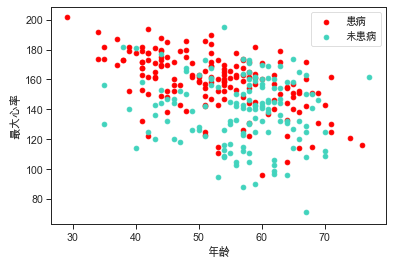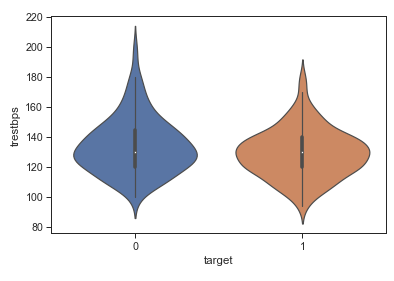看到30岁心跳200那个点，吓我一跳，如果心脏病不是病，那200这个速度太让人膜拜了。

可以看到的是心跳速度患病的大概集中在140-200bpm之间。这个数据比未患病的人普遍高一些，从提琴图上也可以看到这个值分布比健康人高一些且更集中。

## 年龄和血压（trestbps）分布关系

大家都知道体检的时候血压是常规测试项目，那么我想血压和年龄有什么关系吗？有没有心脏病和年龄有关系吗？

来做个图看一下。并尝试用不同的颜色区分。

plt.scatter(x=data.age[data.target==1], y=data.trestbps[data.target==1], c="#FFA773")
plt.scatter(x=data.age[data.target==0], y=data.trestbps[data.target==0], c="#8DE0FF")
plt.legend(["患病",'未患病'])
plt.xlabel("年龄")
plt.ylabel("血压")
plt.show()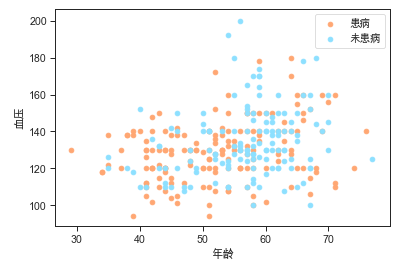看上去随着年龄增长，血压更飘了？从这个结果可以看到的是，静息血压患病人和未患病的人在血压方面都是均匀分布的，随着年龄增长也没有明显的分层变化。所以并不能直接从静息血压很好的判断出是否患心脏病。

那么血压与其他什么有关呢？

比如心率？好，来看看。

## 血压（trestbps）和心率（thalach）关系

血压、心率这两个都来自于心脏的动能，相当于发动机力量和发动机转速。我猜这俩有点关系，一起看看

plt.scatter(x=data.thalach[data.target==1], y=data.trestbps[data.target==1], c="#FFA773")
plt.scatter(x=data.thalach[data.target==0], y=data.trestbps[data.target==0], c="#8DE0FF")
plt.legend(["患病",'未患病'])
plt.xlabel("心率")
plt.ylabel("血压")
plt.show()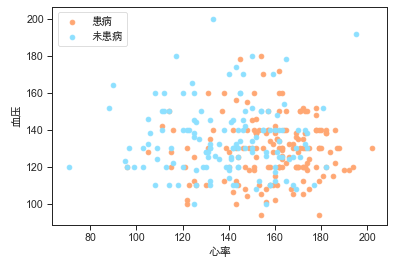现实情况是，这个样本集中，除了能显示出患病新率高这个已有结果外，血压和心率没有相关性。

## 胸痛类型和心脏病、血压三者关系

表中有个数据是胸痛类型四个，分别是0123，他们和心脏病有关系吗，作图看看。

此外这块我要说的是，我上边的翻译是1 典型、2非典型、3非心绞痛、4无症状。

但是数据集中是0123 ，我再kaggle里看了很多人的作品，没有合理解释这个的，所以这个数据我只可视化展示，不分析。

sns.swarmplot(x='target',y='trestbps',hue='cp',data=data, size=6)
plt.xlabel('是否患病')
plt.show()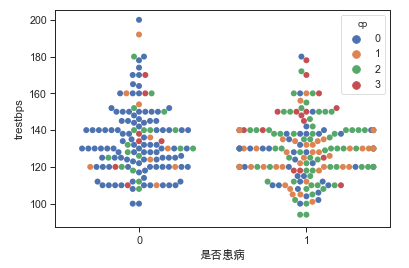fig,ax=plt.subplots(1,2,figsize=(14,5))
sns.countplot(x='cp',data=data,hue='target',palette='Set3',ax=ax)
ax.set_xlabel("胸痛类型")
ax.set_title("胸痛类型")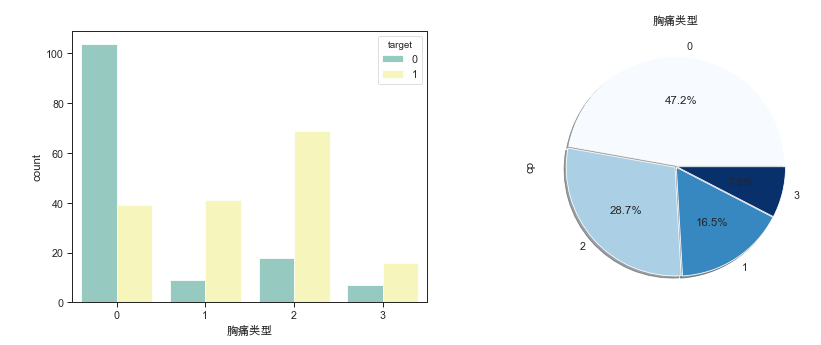结论是：从上图可以看到的是0类疼痛的人在非患病群体中占大多数，而在患病群体中，123三种胸痛的人占了大部分。

## 运动引起的心绞痛与患病、心率关系

承接胸痛类型，运动引起心绞痛与是否患病有没有关系呢？与心率有没有关系呢？作图看一下

PS:运动引起心绞痛（exang: 1=有过；0=没有）

sns.swarmplot(x='exang',y='thalach',hue='target',data=data, size=6)
plt.xlabel('有没有过运动引起心绞痛')
plt.ylabel('最大心率')
plt.show()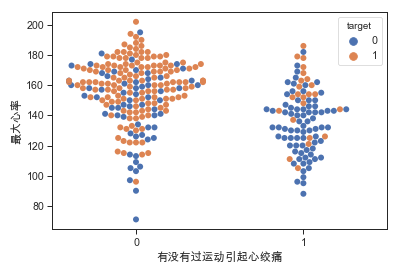得到的这个图像很有意思！

虽然最大心率是入院时候测的，但是在没有运动引起心绞痛的人中，最大心率集中度比较高，在160-180之间，而他们都患有心脏病。

我推测是：他们有心脏病，运动就难受，所以就不运动，所以根本不会有“运动时产生胸痛”这种问题。

而在运动中产生胸痛的人中（右边为1的）他们有很多产生过胸痛，这种人心率比较高，在120-150之间集中着，而其中很多人并没有心脏病，只是心率比较高。

## 大血管数量（ca）和血压（trestbps）、患病关系

plt.figure(figsize=(15,5))
sns.swarmplot(y='trestbps',data=data,x='ca',hue='target',palette='RdBu_r',size=7)
plt.xlabel('大血管数量')
plt.ylabel('静息血压')
plt.show()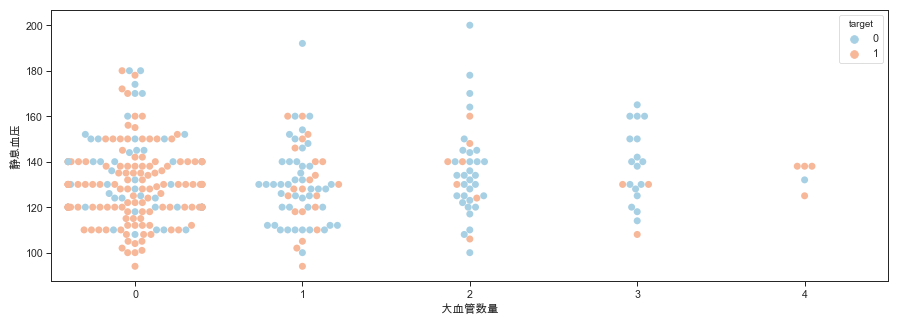plt.figure(figsize=(15,5))
sns.catplot(x="ca", y="age", hue="target", kind="swarm", data=data, palette='RdBu_r')
plt.xlabel('大血管显色数量')
plt.ylabel('年龄'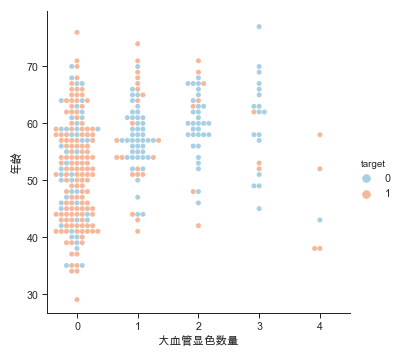这个血管数量指银光显色。具体医学含义没搜到，所以不分析。只是为0的和患病有很大的相关性

## 年龄（age）和胆固醇（chol）关系

在我初高中的时候，我妈妈告诉我说，每天鸡蛋黄不要超过两个，不然会引起胆固醇高，那时候身体健康，从来不信这些话。我后来上大学了连每天一个都没保证住，但我记住了这句话，所以看到胆固醇三个字会想起这个家庭教育哈哈。

胆固醇侧面反映了血脂，那么下边生成一下胆固醇、年龄、患病三者关系散点图。为了区分，这次我又换了个颜色。

plt.scatter(x=data.age[data.target==1], y=data.chol[data.target==1], c="orange")
plt.scatter(x=data.age[data.target==0], y=data.chol[data.target==0], c="green")
plt.legend(["患病",'未患病'])
plt.xlabel("年龄")
plt.ylabel("胆固醇")
plt.show()
# 箱型图
sns.boxplot(x=data.target,y=data.chol,data=data)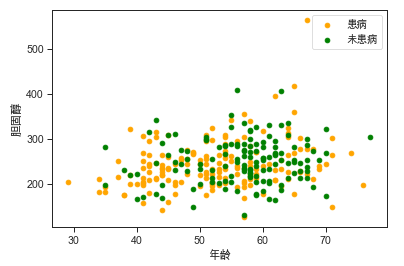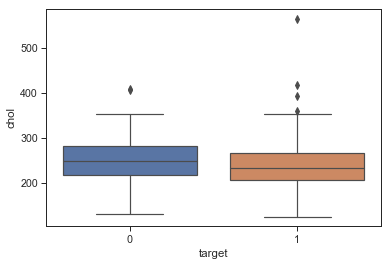在这个样本集中，患病者和非患病者胆固醇含量分布没有明显的分层现象，箱型图显示结果是合理上下限是一样的，只是25%、50%、75%三条线患病的人稍微稍微低一些。

结论就是胆固醇并不能直接反映有没有心脏病这件事。

## 相关性分析

分析了很多，那么哪些和患病相关的，而数据间又有啥关系呢？做个图看看,颜色越绿越相关，越红越负相关

plt.figure(figsize=(15,10))
ax= sns.heatmap(data.corr(),cmap=plt.cm.RdYlBu_r , annot=True ,fmt='.2f')
a,b =ax.get_ylim()
ax.set_ylim(a+0.5,b-0.5)图像很好看对不对，只看最后一行，是否患病和cp、thalach、slope正相关，和exang、oldpeak、ca、thal等负相关。

本篇分析了心脏病数据集中的部分内容，14列其实有非常多的组合方式去分析。此外本文没有用到模型，只是数据可视化的方式进行简要分析。

本文中由于图片过大，在手机浏览可能看不清楚，故开源了代码，欢迎大家自己动手可视化试试。

如果有什么建议意见，欢迎留言。

获取本文涉及的源码和数据集：后台回复“心脏病

------------------- End -------------------

往期精彩文章推荐：

• ## 手把手用Python网络爬虫带你爬取全国著名高校附近酒店评论欢迎大家点赞，留言，转发，转载，感谢大家的相伴与支持

想加入Python学习群请在后台回复【入群

万水千山总是情，点个【在看】行不行

/今日留言主题/

随便说一两句吧~~

展开全文• 数据集包含14个属性，分类的target为是否有心脏，分别由0，1表示。 属性包括： age 年龄 sex 性别 chest pain type 胸痛的类型 resting blood pressure 静息血压 serum cholestoral in mg/dl 血清...
• 目录 一、理论知识回顾 1、神经网络模型 2、明确任务以及参数 ...2、数据准备、数据处理、数据划分 1）数据下载 2）关键代码 3、初始化待估参数 1）关键代码 2）np.random.randint(a,b,(m,n))函数神经网络 算法 python 机器学习 深度学习
• ## 数据分析实战

千次阅读 2021-03-23 15:14:35
数据分析实战数据分析基础数据分析全景图及修炼指南学习数据挖掘的最佳路径学数据分析要掌握哪些基本概念用户画像：标签化就是数据的抽象能力数据采集：如何自动化采集数据数据采集：如何用八爪鱼采集微博上的“D&...大数据
• 我们都很害怕生病，但感冒发烧这种从小到大的疾病我们已经麻木了，因为一星期他就会好，但是随着长大，各种发炎、三高、心脏、冠心病响应而生。 心脏作为一种发作起来...今天在kaggle上看到一个心脏病数据（...
• 计算精确率、召回率以及f-分数 【思考】什么是二分类问题的混淆矩阵，理解这个概念，知道它主要是运算到什么任务中的 #混淆矩阵是一个 2 维方阵，它主要用于评估二分类问题（例如：预测或未心脏、股票涨或跌等...
• 数组是数据分析的基础，使用数组需要先加载两个包 Numpy 和 Pandas包一、一维数组数据分析在一维数组中更实用的的是pandas的Serise数组功能，numpy主要应用于值计算，两种数组都具有查询功能，向量化运算。...
• 大数据背景下，基于对数据挖掘和运用基础上的智慧城市建设是城市发展的必然趋势，是新时期实现城市科学发展、高效管理与公共服务更优化的重要战略。政府作为社会管理、城市治理的主体，其形成、管理的数...大数据 人工智能 makefile
• 数据分析的步骤一般可以分为6个：1，明确分析的目的2，数据准备3，数据清洗4，数据分析5，数据可视化6，分析报告数据分析的目的：通过对朝阳区医院的药品销售数据的分析，了解朝阳医院的患者的月均消费次数，月均...
• ## 医学图像数据集汇总

万次阅读 多人点赞 2020-01-15 12:20:30
• 实验名称 实验一 单链表的基本操作 实验目的 1．理解线性表的逻辑结构； 2．理解单链表的存储结构特点，掌握单链表...（5）在位置i插入一个数据元素； （6）删除位置i的数据元素； （7）遍历单链表； （...算法
• 写在前面 从省向下查三级, 高德县级市的子级会返回街道，正常的市正常返回子级为区。 具体高德api参数详情请查看...代码 //获取json数据 public function getDataAction() { set_time_limit(0); \$url="...
• 数据集的目标是基于数据集中包含的某些诊断测量来诊断性的预测 患者是否有糖尿病。从较大的数据库中选择这些实例有几个约束条件。尤其是，这里的所有患者都是印第安至少21岁的女性。数据集由多个医学预测变量和一...
• 中国北京，2017年8月7日，可视化分析领导者Qlik®今天宣布，新西兰最大的私人医院 MercyAscot 采用 Qlik可视化分析平台来提高病患护理质量。通过高效地对数据进行可视化处理和分析，MercyAscot 现在能够更准确的确定...
• MIMIC数据库数据提取教程：如何提取患者首次入ICU信息。数据库
• 在近几十年内，电子医疗记录（EHR）的普及为科学研究带来了数百万病患的详细医疗数据。大量的数据使利用机器学习模型对病人做出个性化的预测成为可能。 传统方法将病人的生存预测视作一个概率分类问题，即在一定的...
• 15、在某座城市出现了某种流行，且已知某座城市男女人数相同，男性的概率为5%，女性为0.25%，现已知该城市的小明得了此，请问小明为男性的概率是（） B A、5% B、95% C、40% D、60% 解析： 令人是男性为...python sql 机器学习
• 第三届Python数据分析职业技能比赛A题赛题竞赛背景字段说明考核目标任务任务一 数据预处理任务二 数据可视化任务三 数据分析任务一思路任务二思路python
• 糖尿病数据挖掘慢病之王是什么怎么做   慢病之王 国际糖尿病联合会：https://idf.org/ 英国糖尿病组织官网：http://www.diabetes.org.uk/   是什么   怎么做机器学习 人工智能
• 听起来，这有点天方夜谭，毕竟未来的一切都是不可预测的，但是，随着技术的进步，AI预测死亡或许正在变为现实，起码谷歌的研究证明其在病患身上已经凸显作用。 对病人来说，知道自己的死亡时间，无疑是会加大其心理...
• 开放数据是什么？开放数据的好处是什么？医疗卫生领域开放数据的目的是什么？医疗卫生领域开放数据的类型是什么？医疗卫生领域开放数据的有利因素是什么？全球医疗卫生领域开放数据的时间线是怎样的？中国医疗卫生...
• 导读：归纳思维，是从特殊情况出发，推理出一般性的结论，作为数据分析的重要思维，我认为应该引起足够的重视。下面介绍 5 种归纳方法，即：求同法、求异法、共用法、共变法和剩余法，其实这些方法早...人工智能 机器学习 python 编程语言
• 一、数据源训练数据源为LIDC-IDRI，该数据集由胸部医学图像文件(如CT、X光片)和对应的诊断结果病变标注组成。该数据是由美国国家癌症研究所(National Cancer Institute)发起收集的，目的是为了研究高危人群早期癌症...
• ## EEG 公开数据集整理

千次阅读 2021-09-15 09:00:21
许多研究者使用EEG这项技术开展科研工作时，经常会遇到这样一个问题：有很好的idea但苦于缺乏足够的数据支持和验证。尤其是在2019 - 2020年COVID-19期间，许多高校实验室处于封闭状态，不能进入实验室采集脑电数据。...数据库
• 数据分析如何进行预测分析As science gets more advanced, we realise how interconnected our world is. The smallest things can have the biggest impacts. For instance, researchers are today looking at how ...机器学习 python 大数据
• 数据仓库各层表 数据 示例There is a lot of talk about how data, data science, and machine learning can all be applied to help make critical business decisions. 关于如何将数据数据科学和机器学习全部...python...